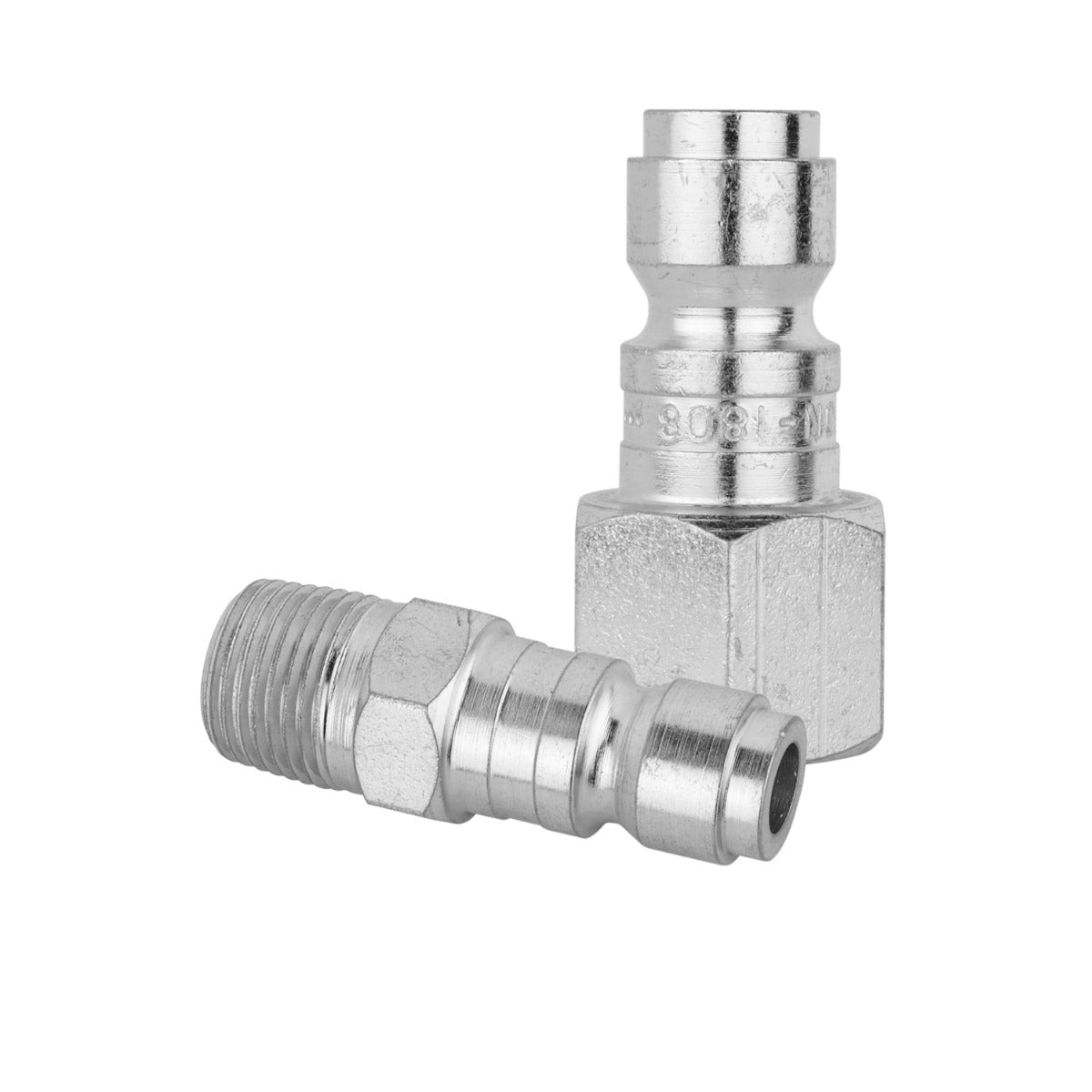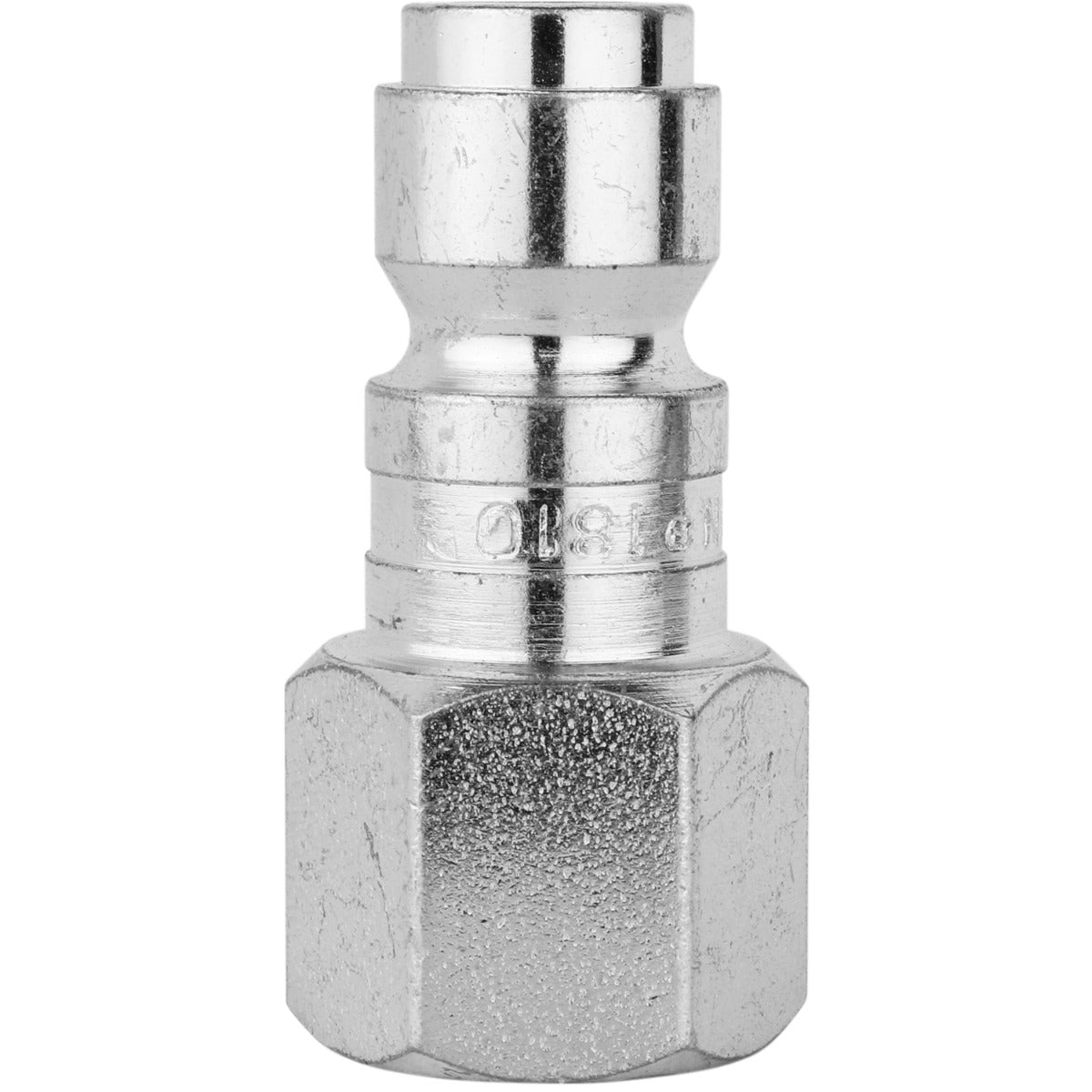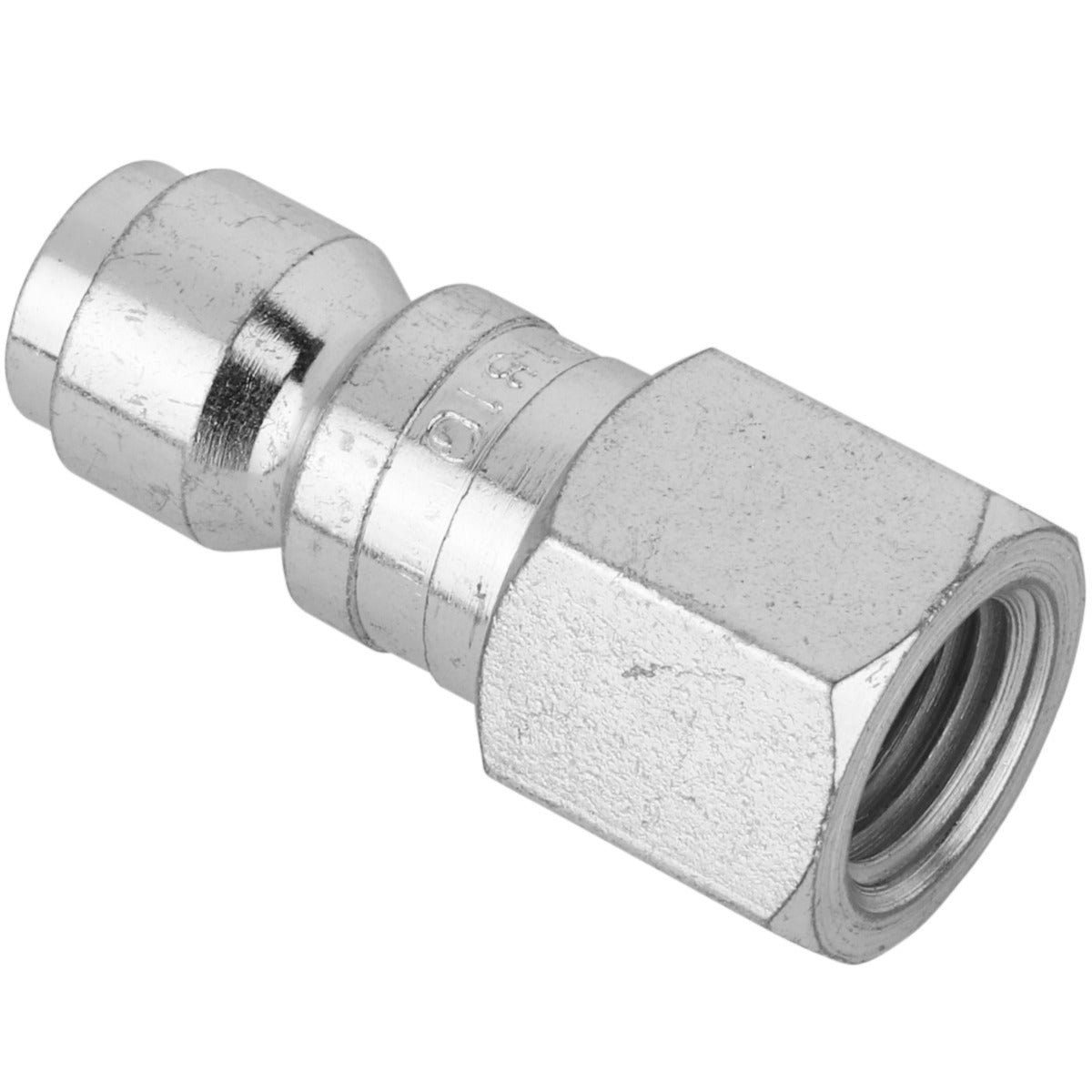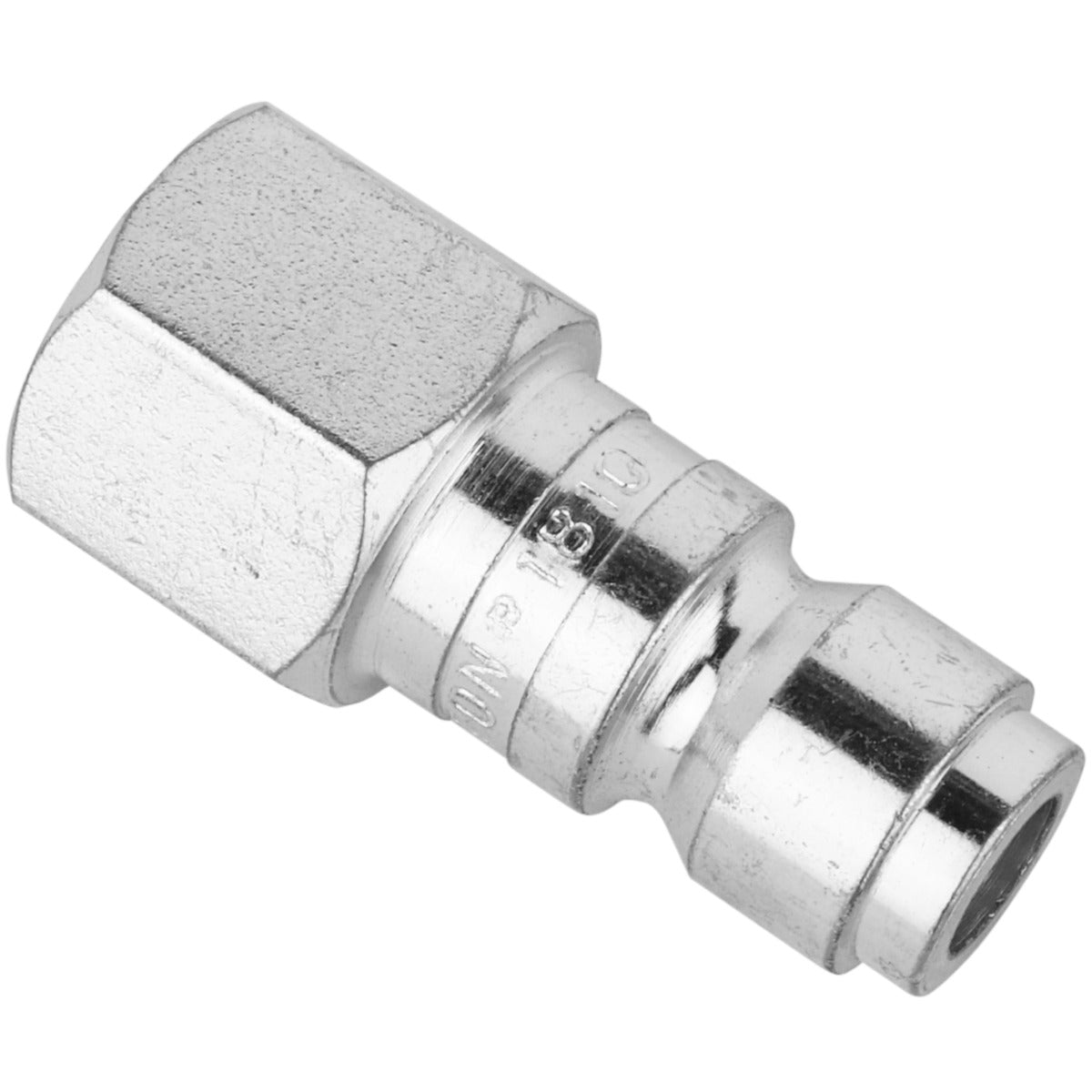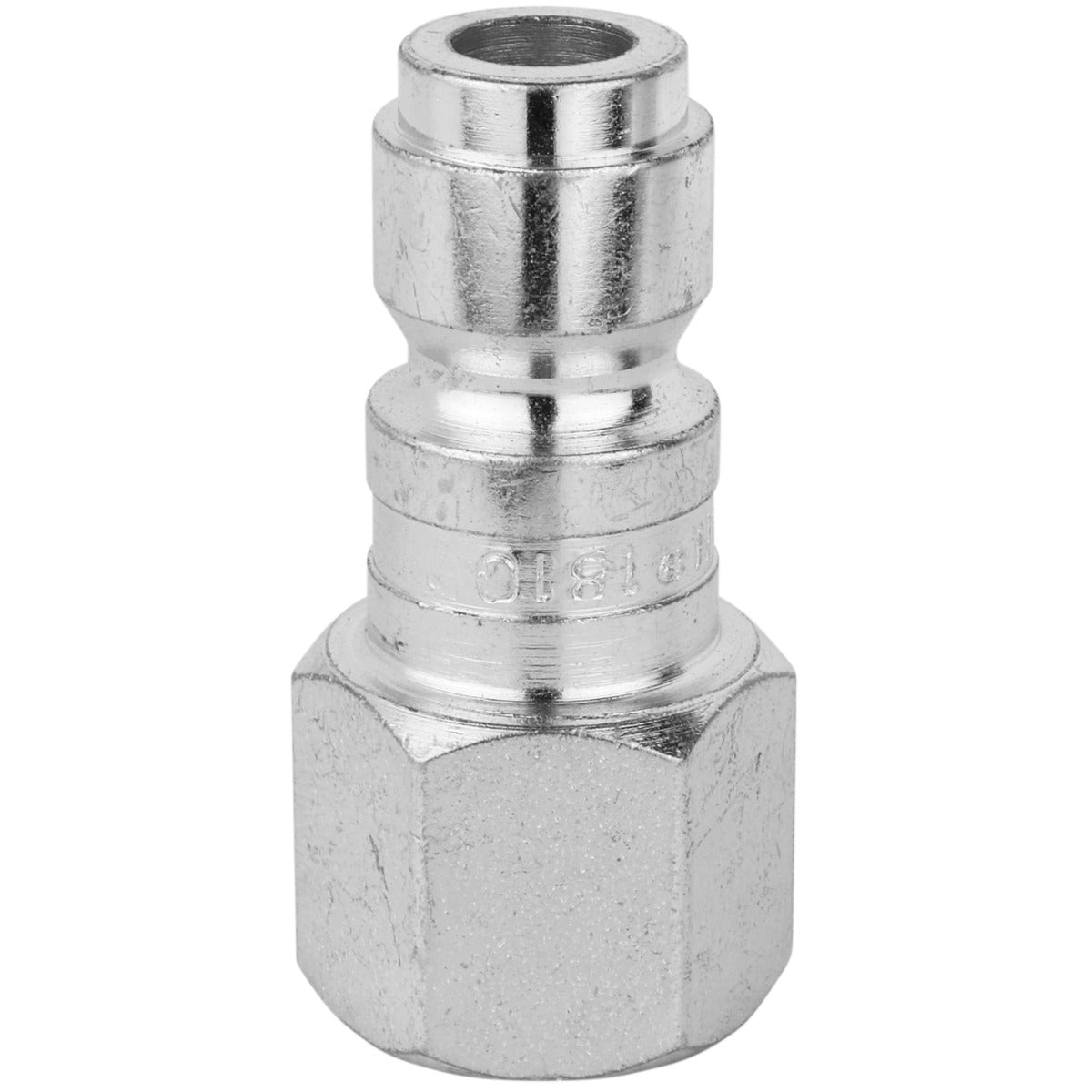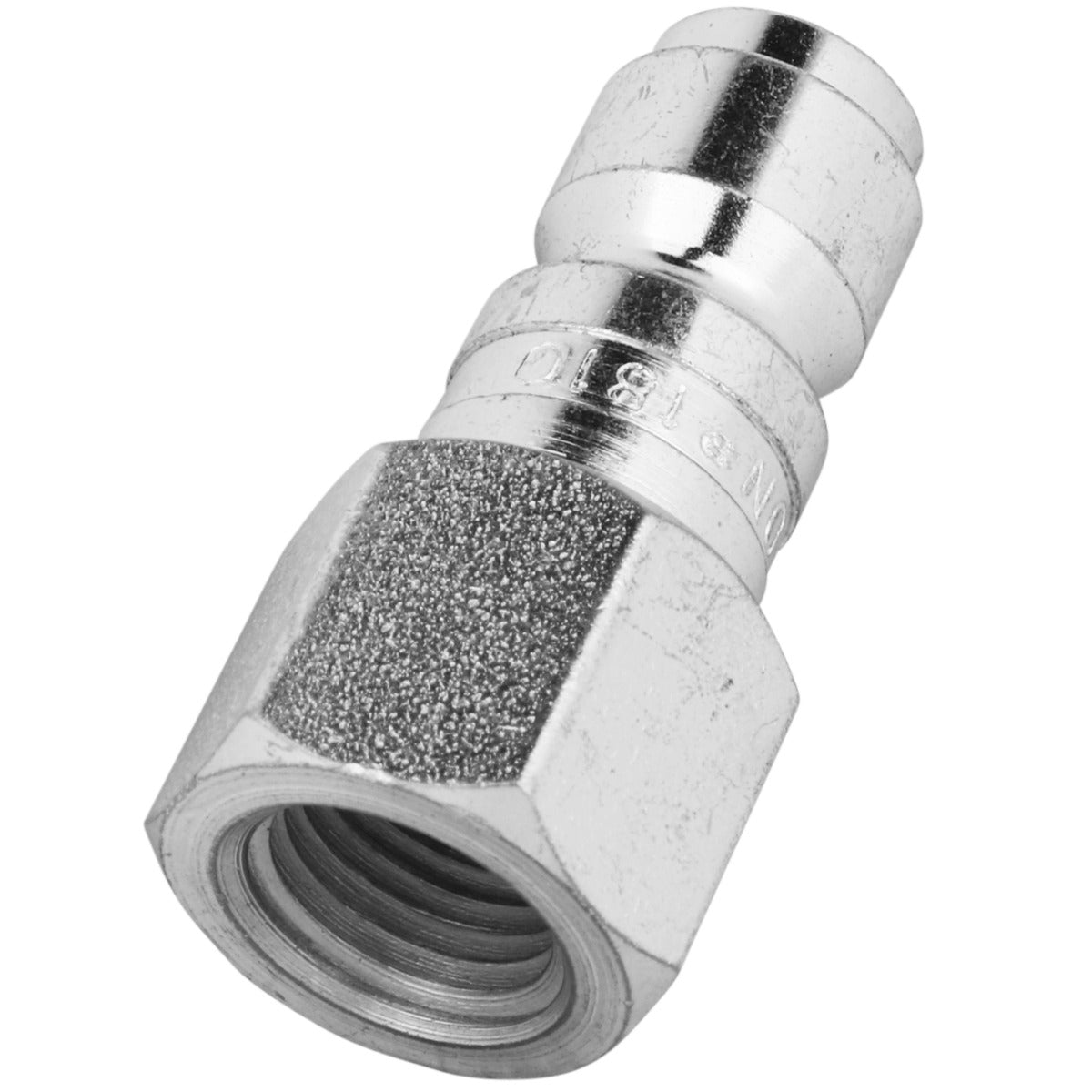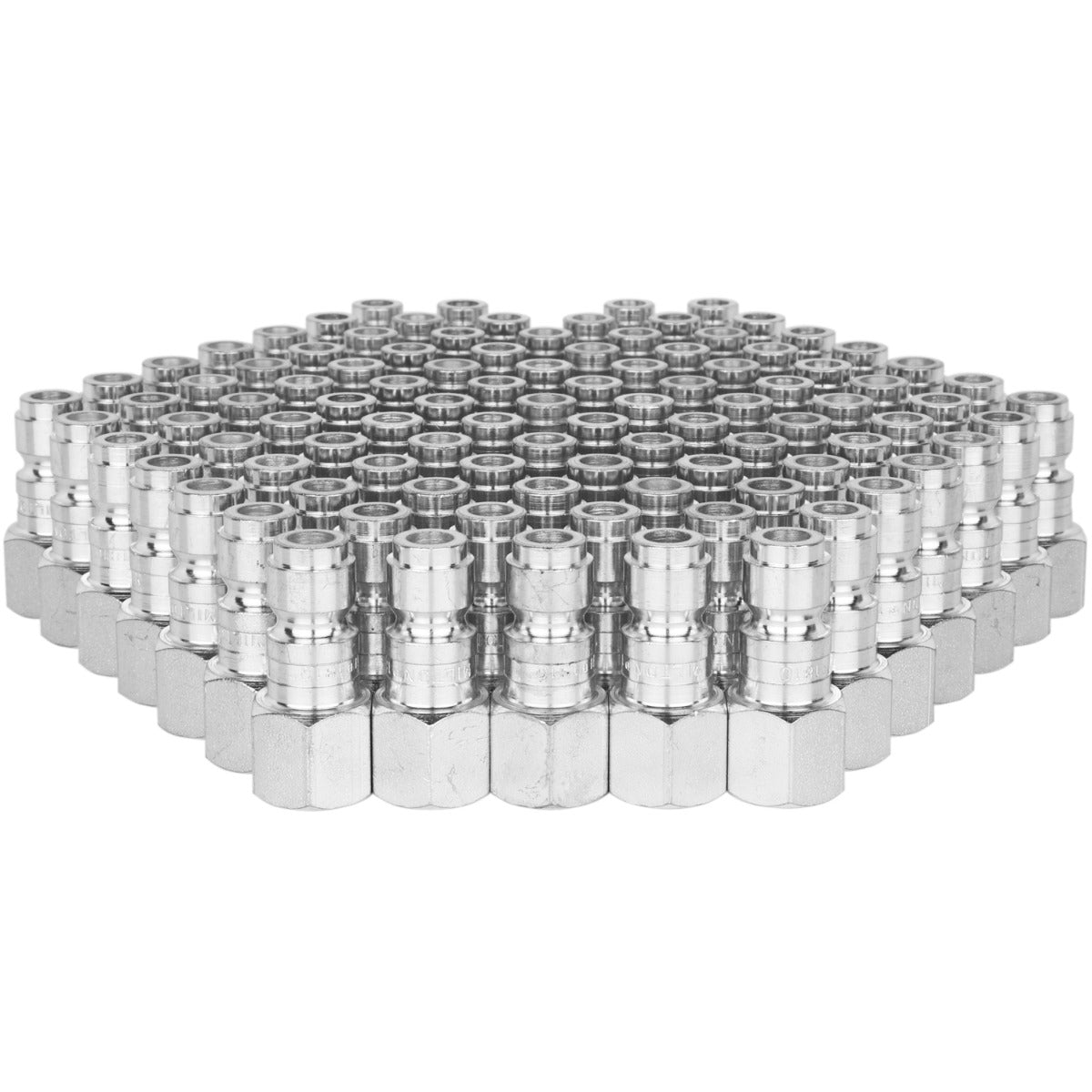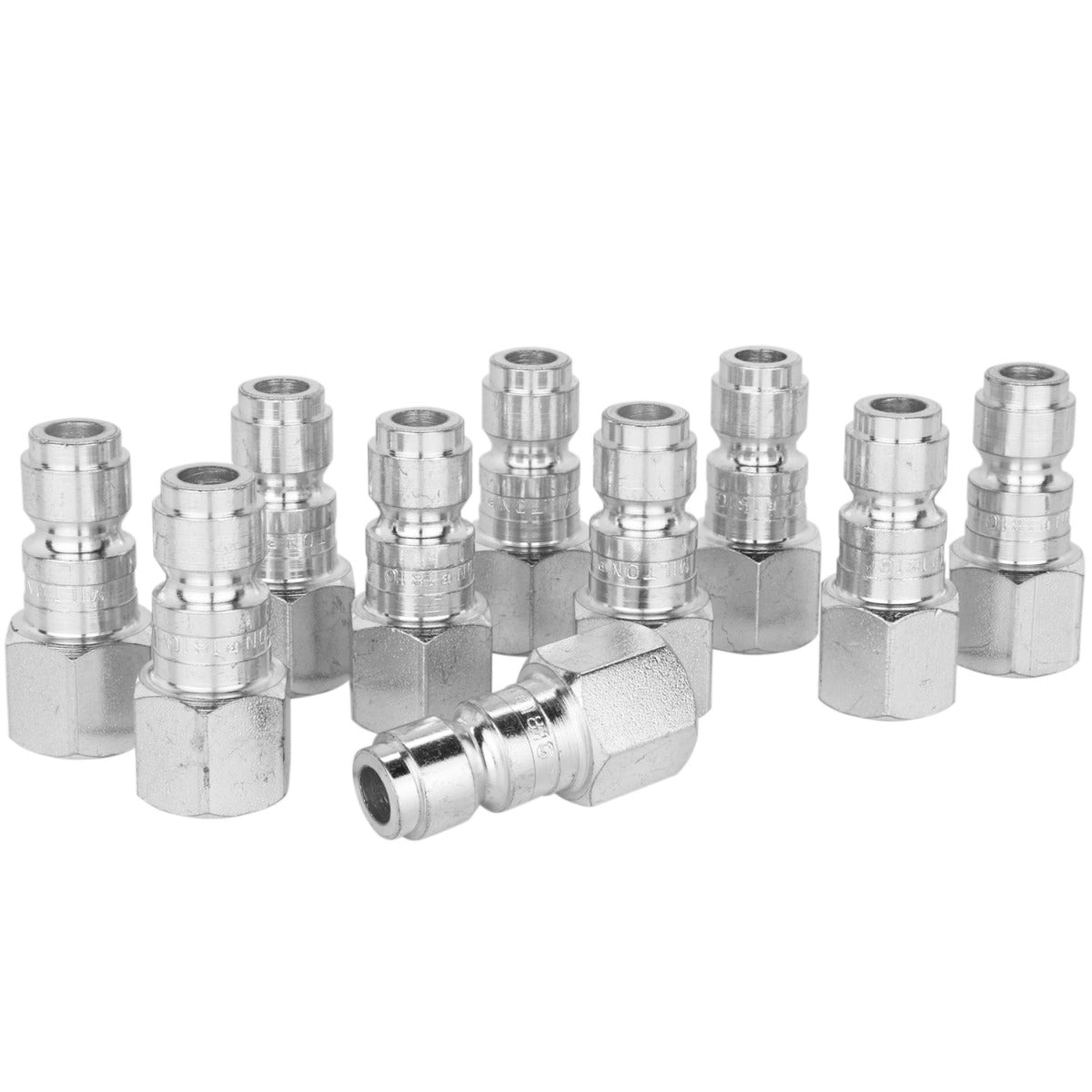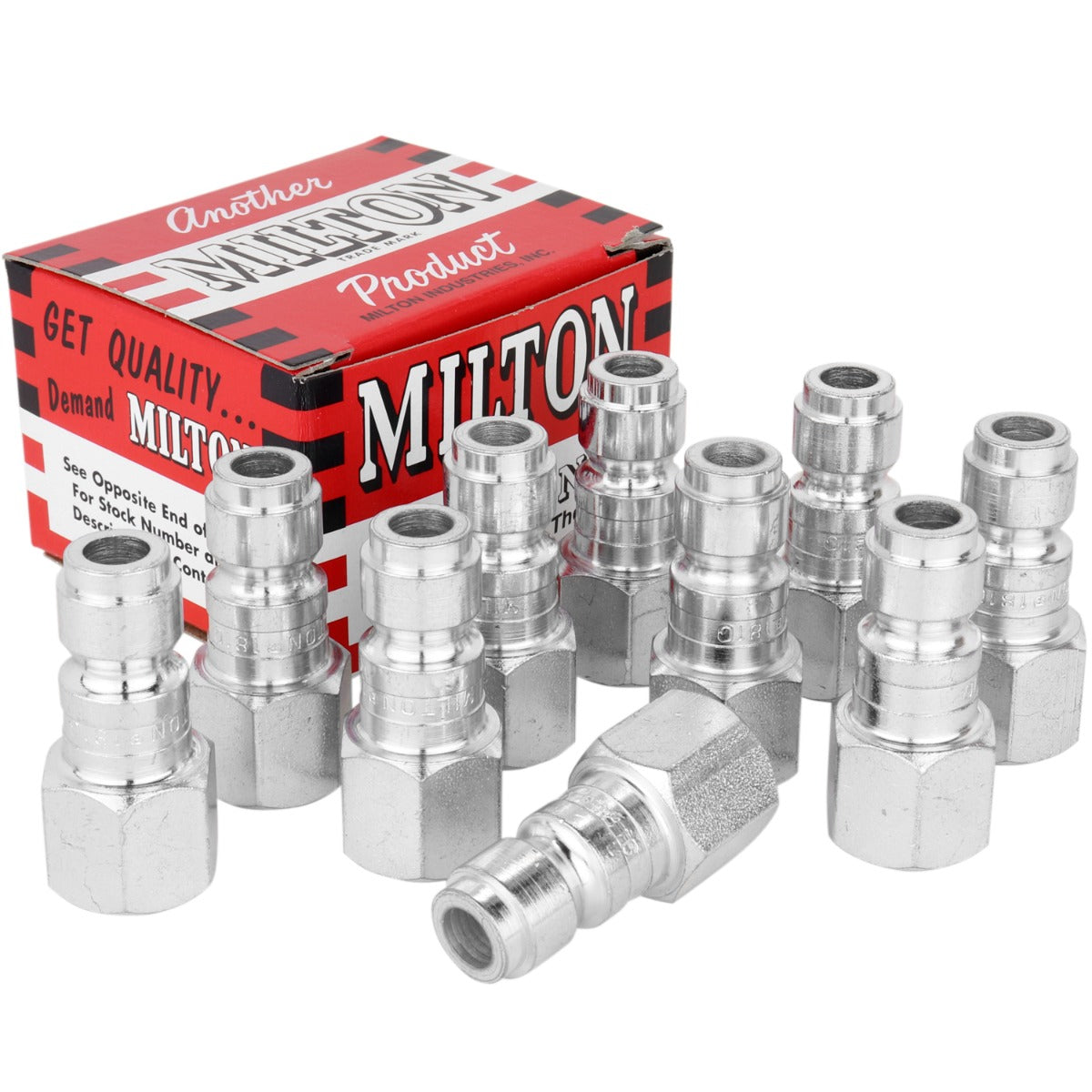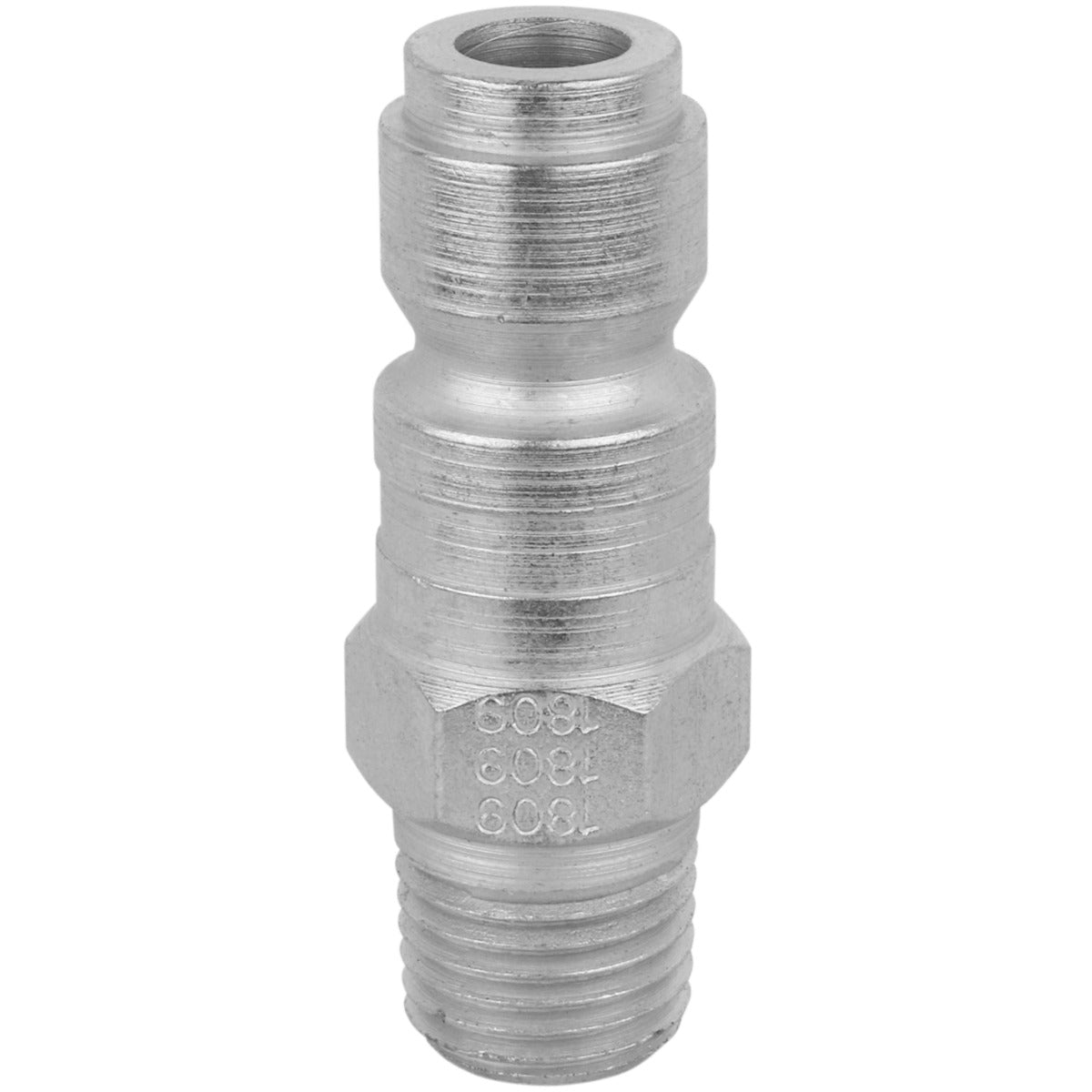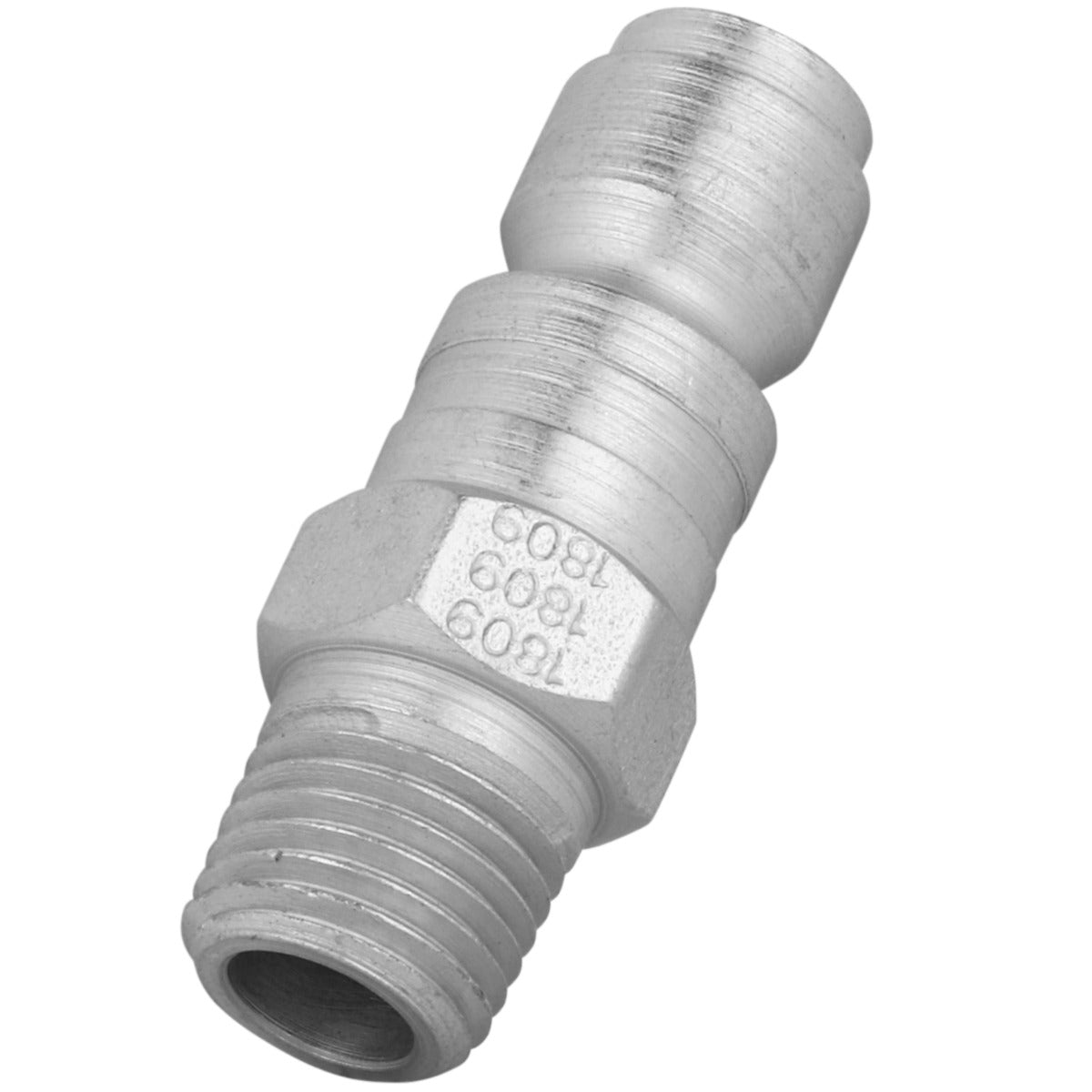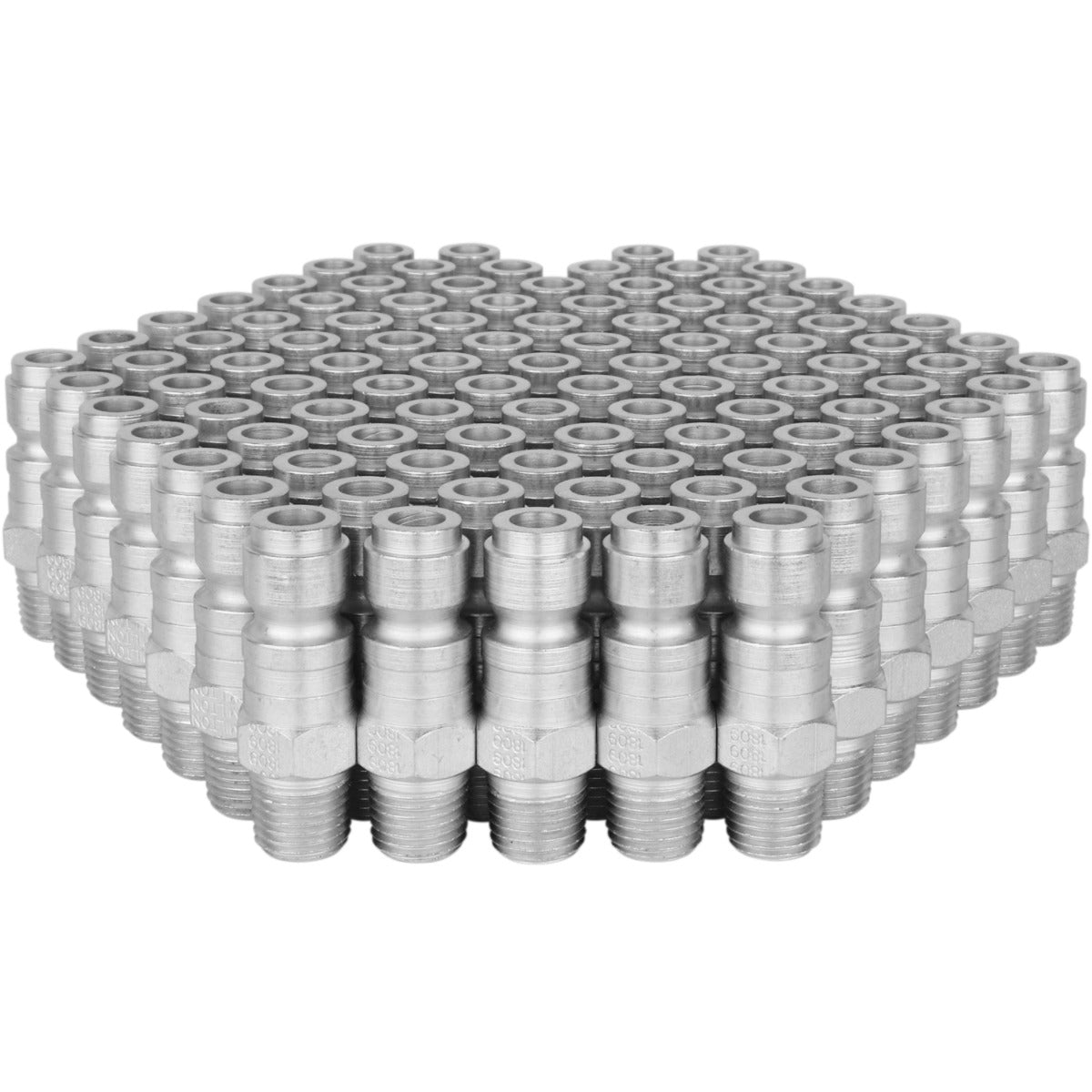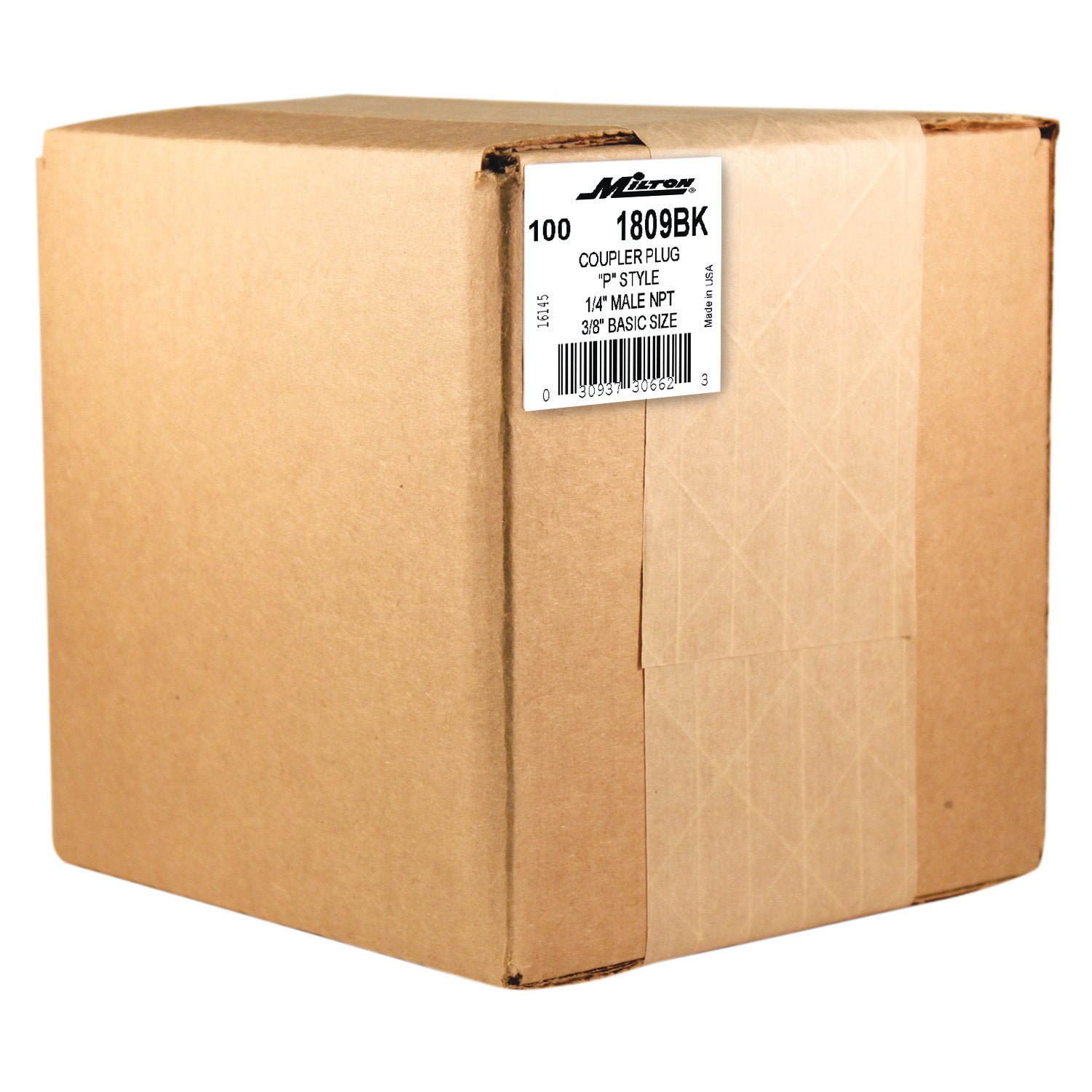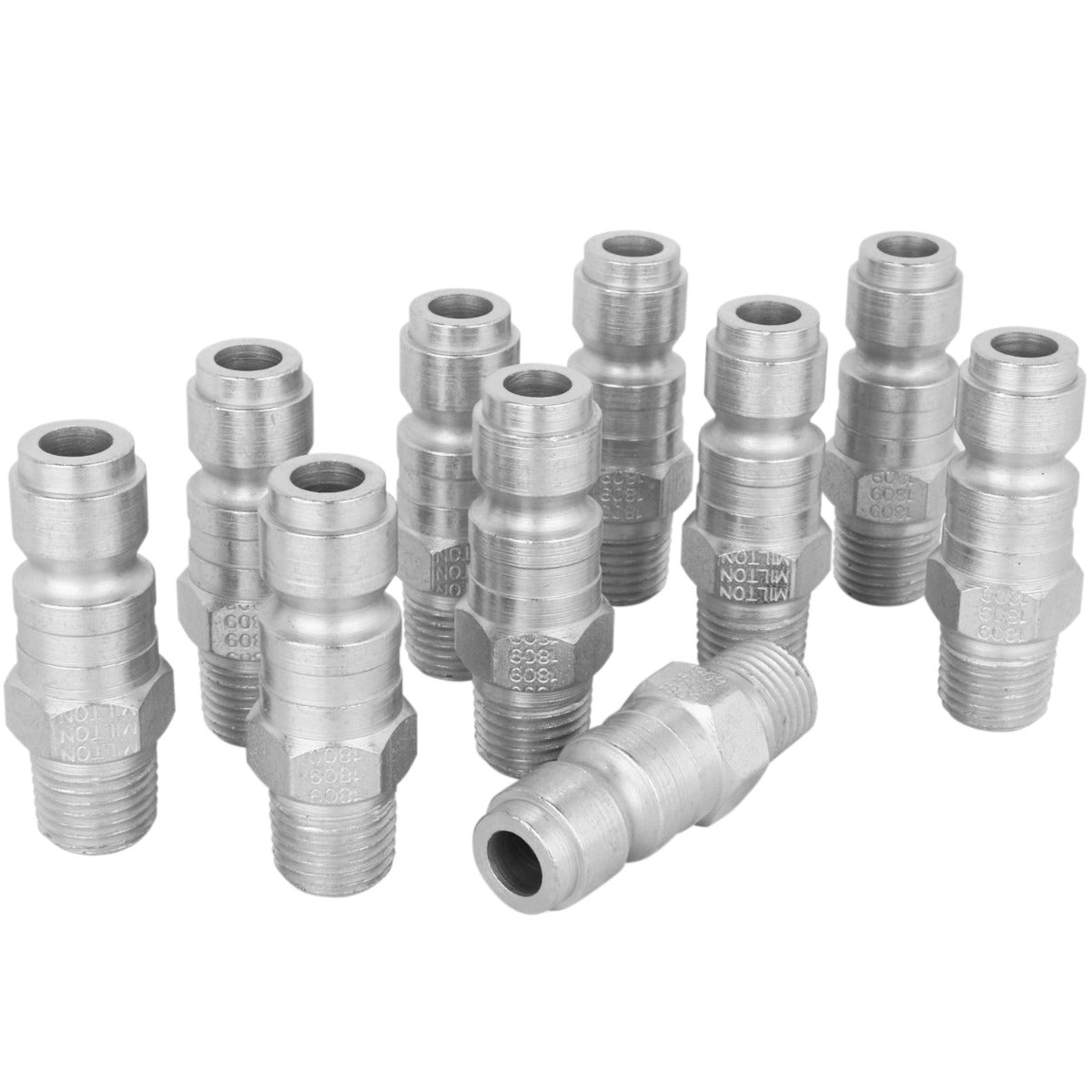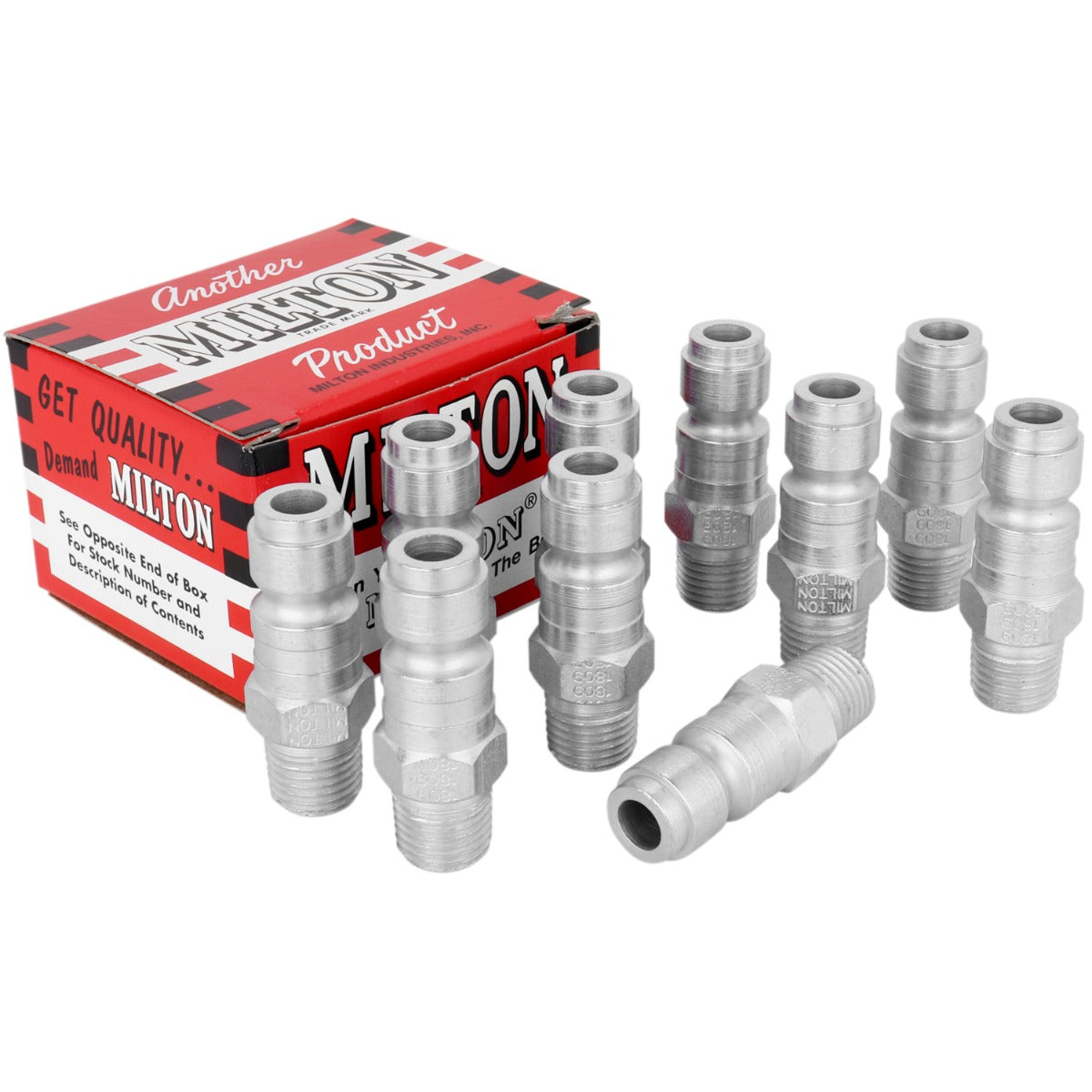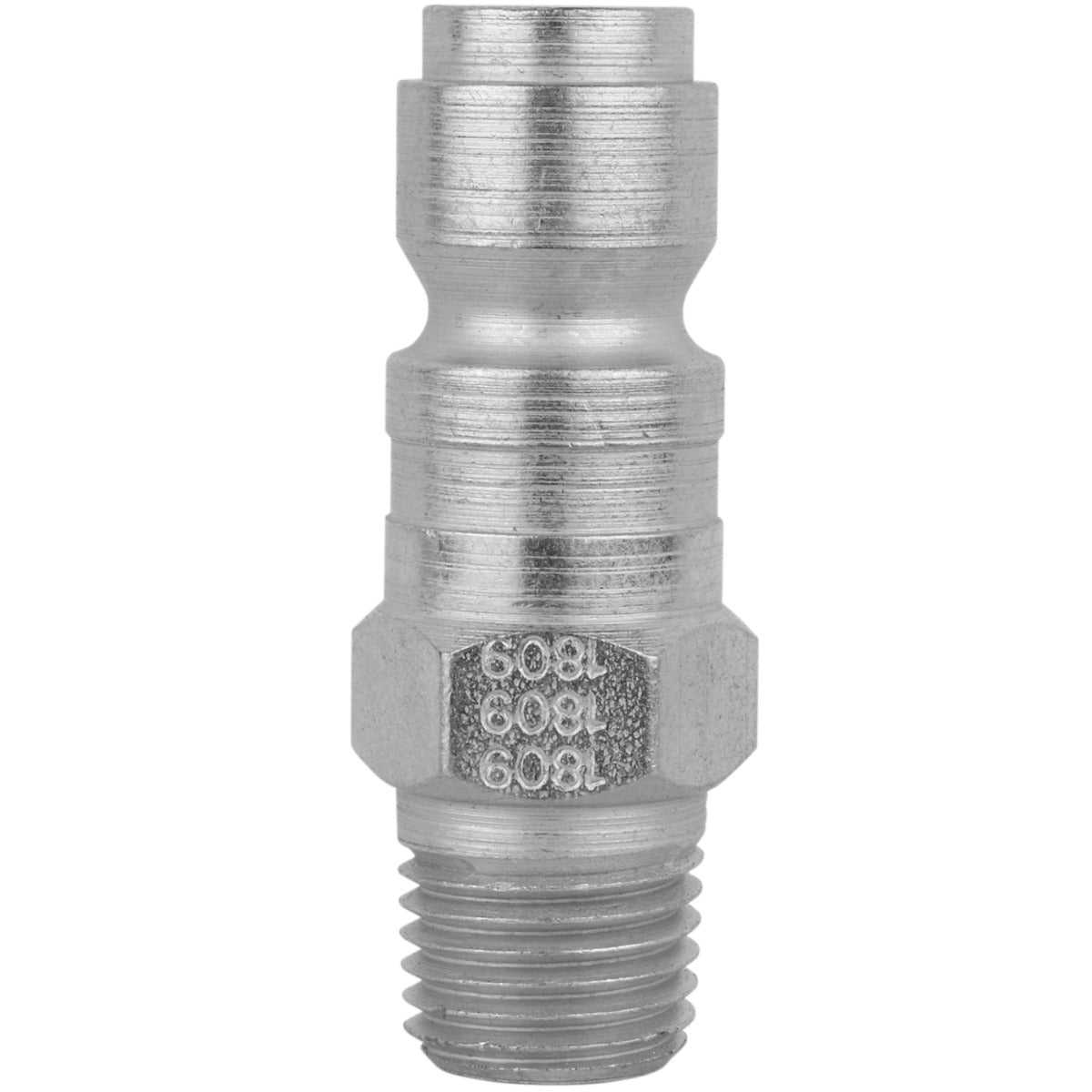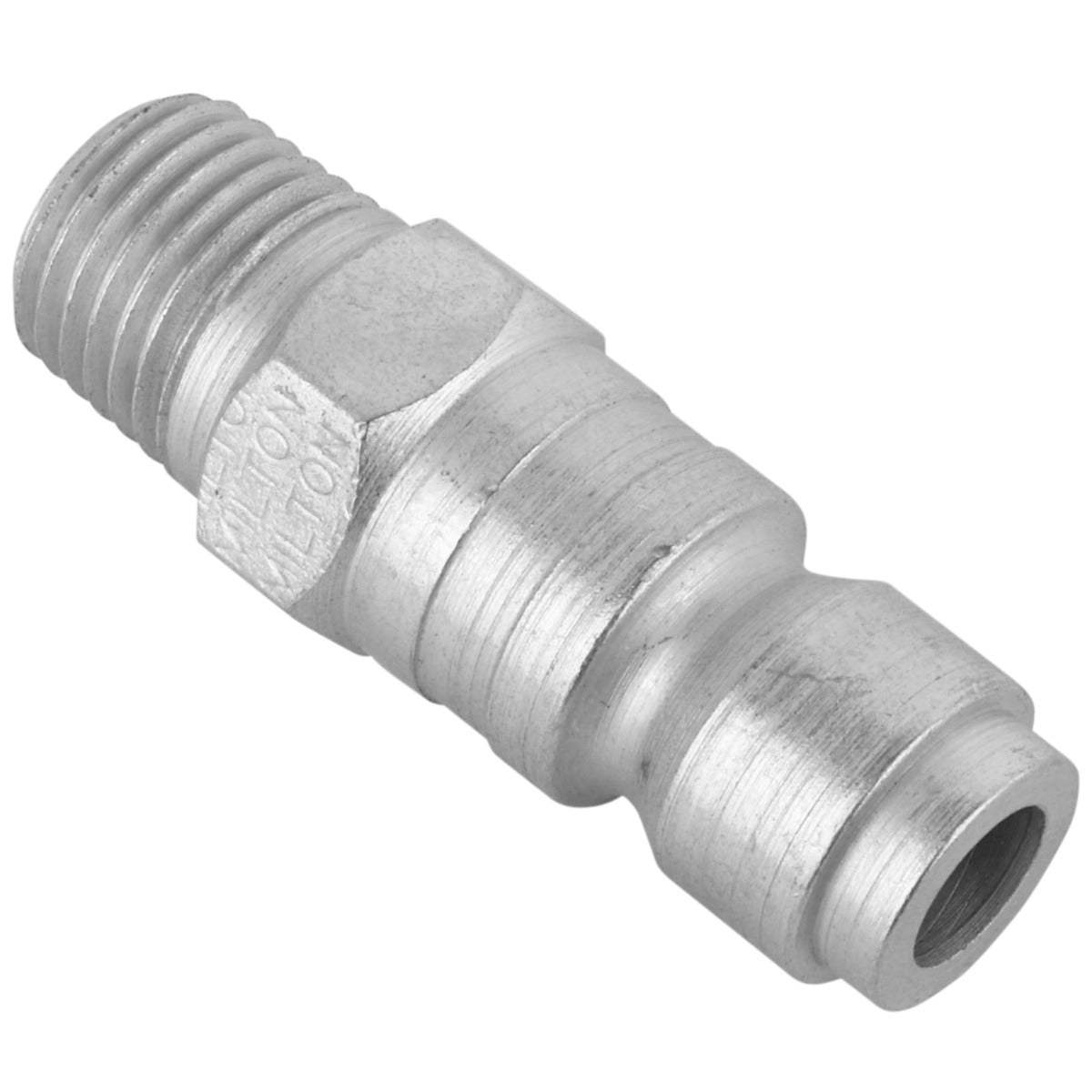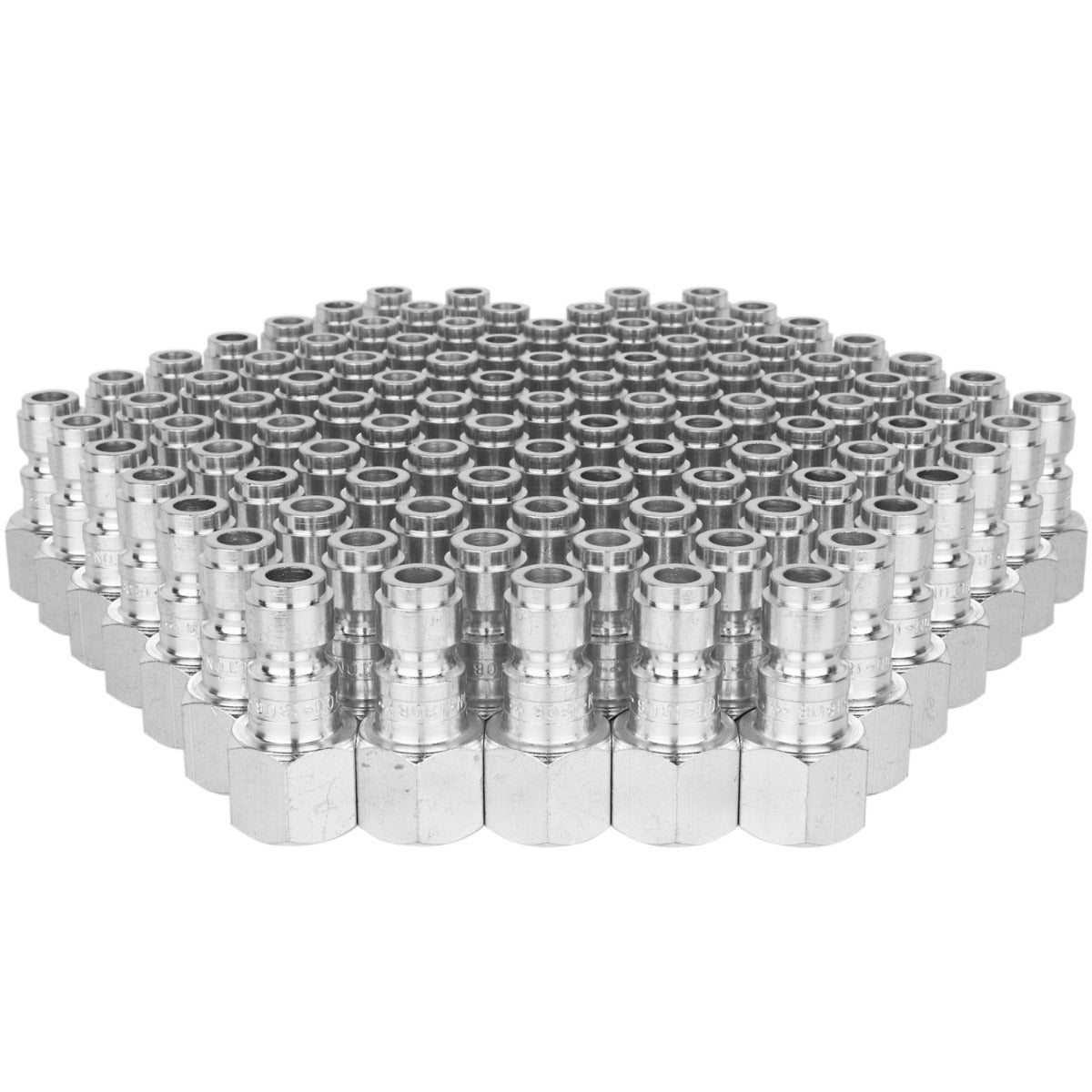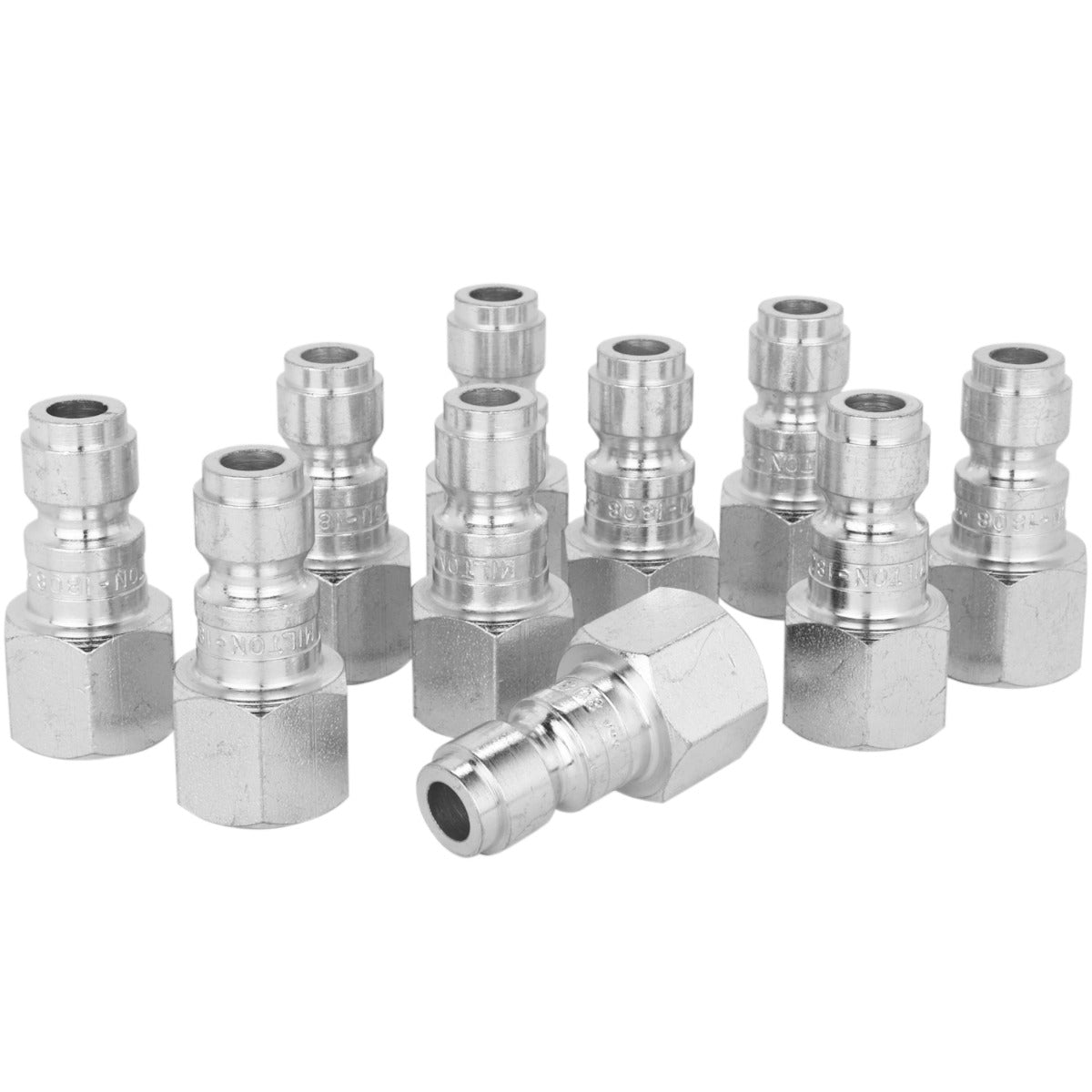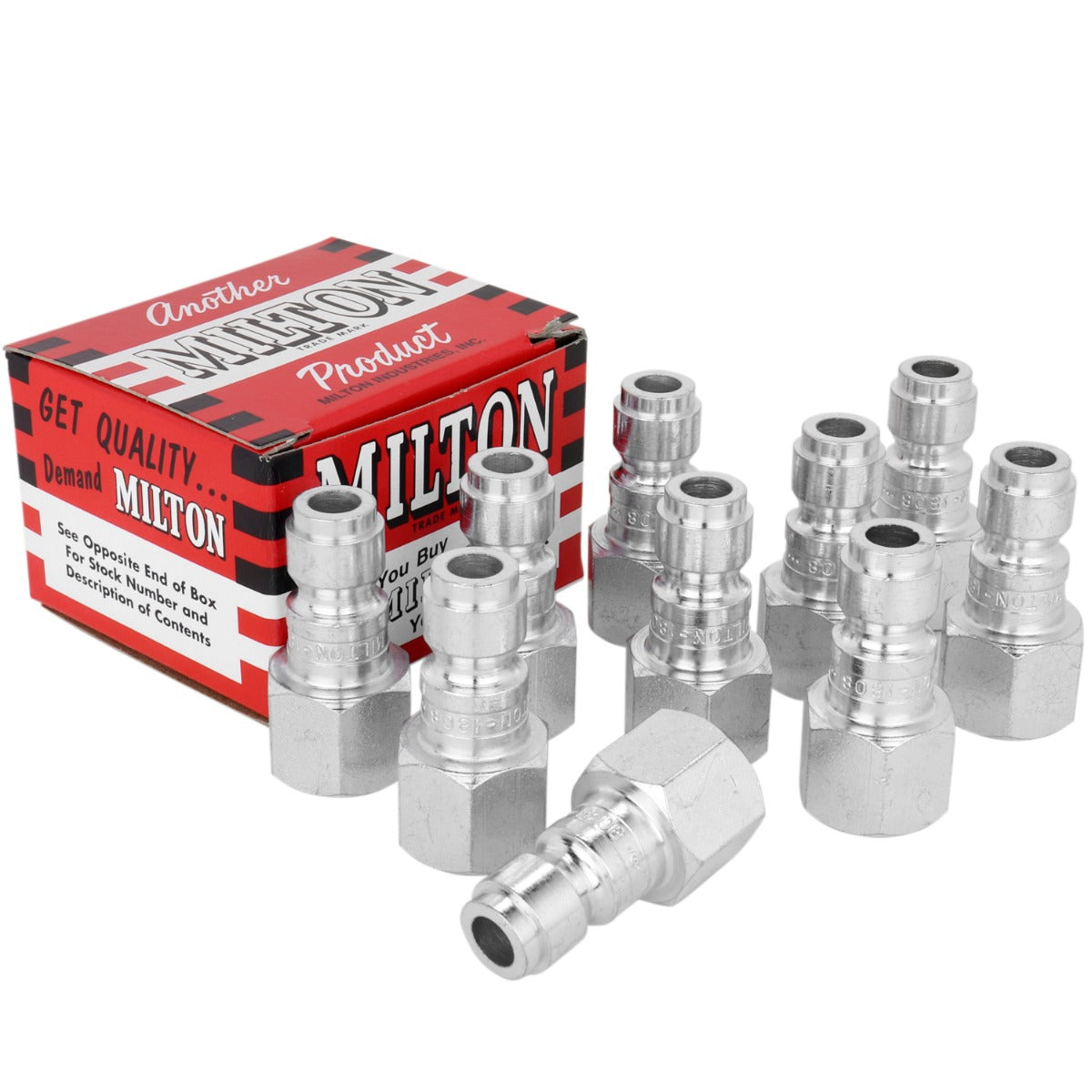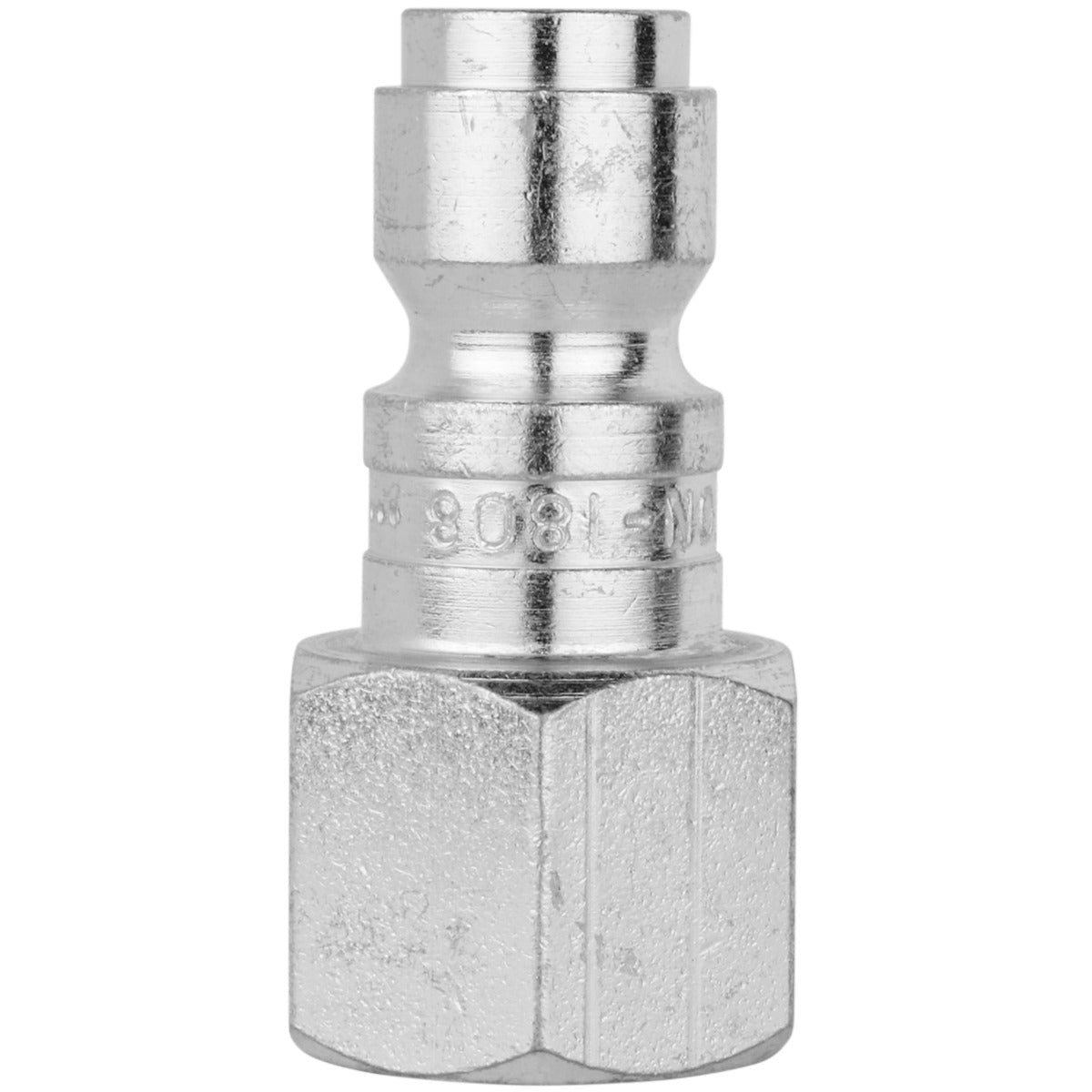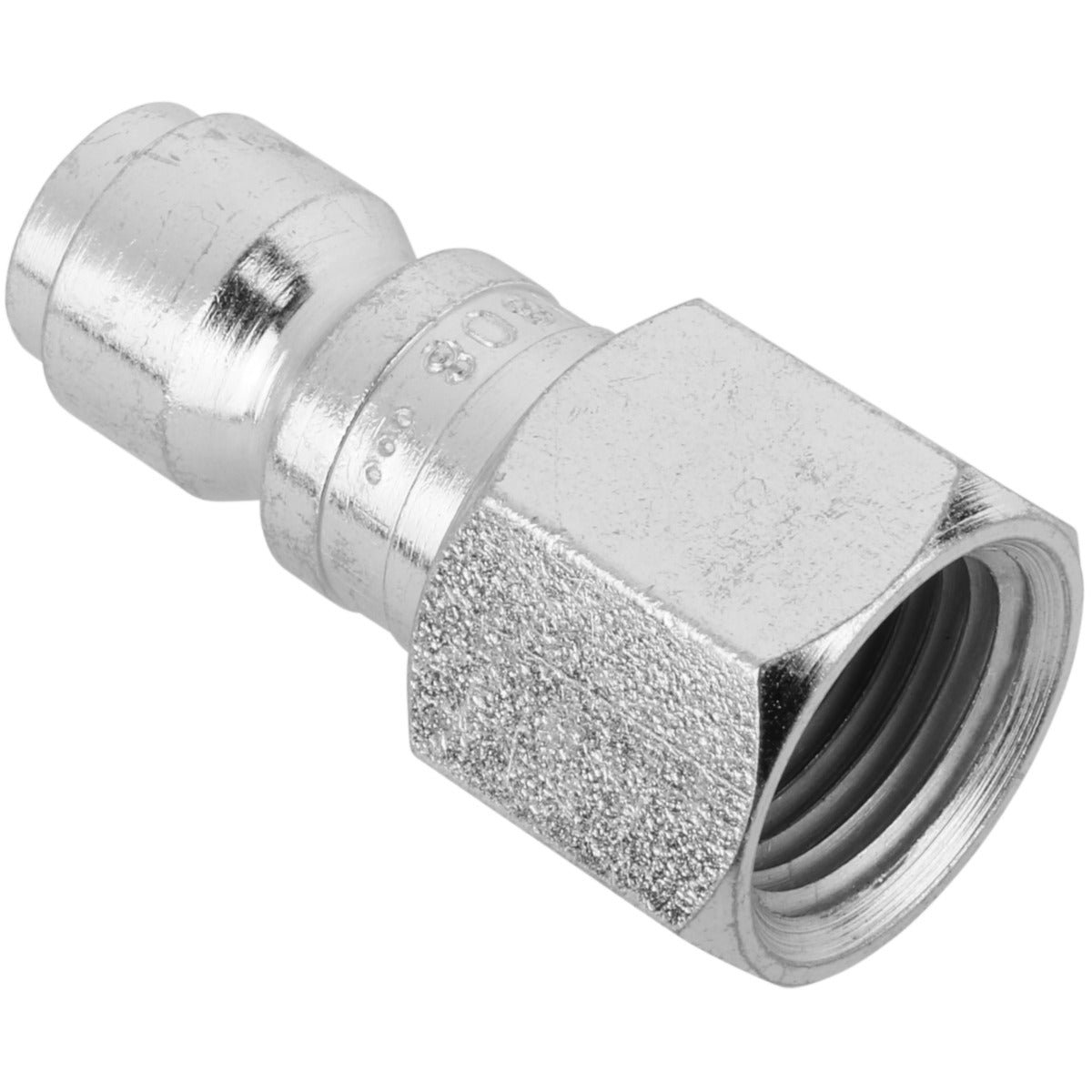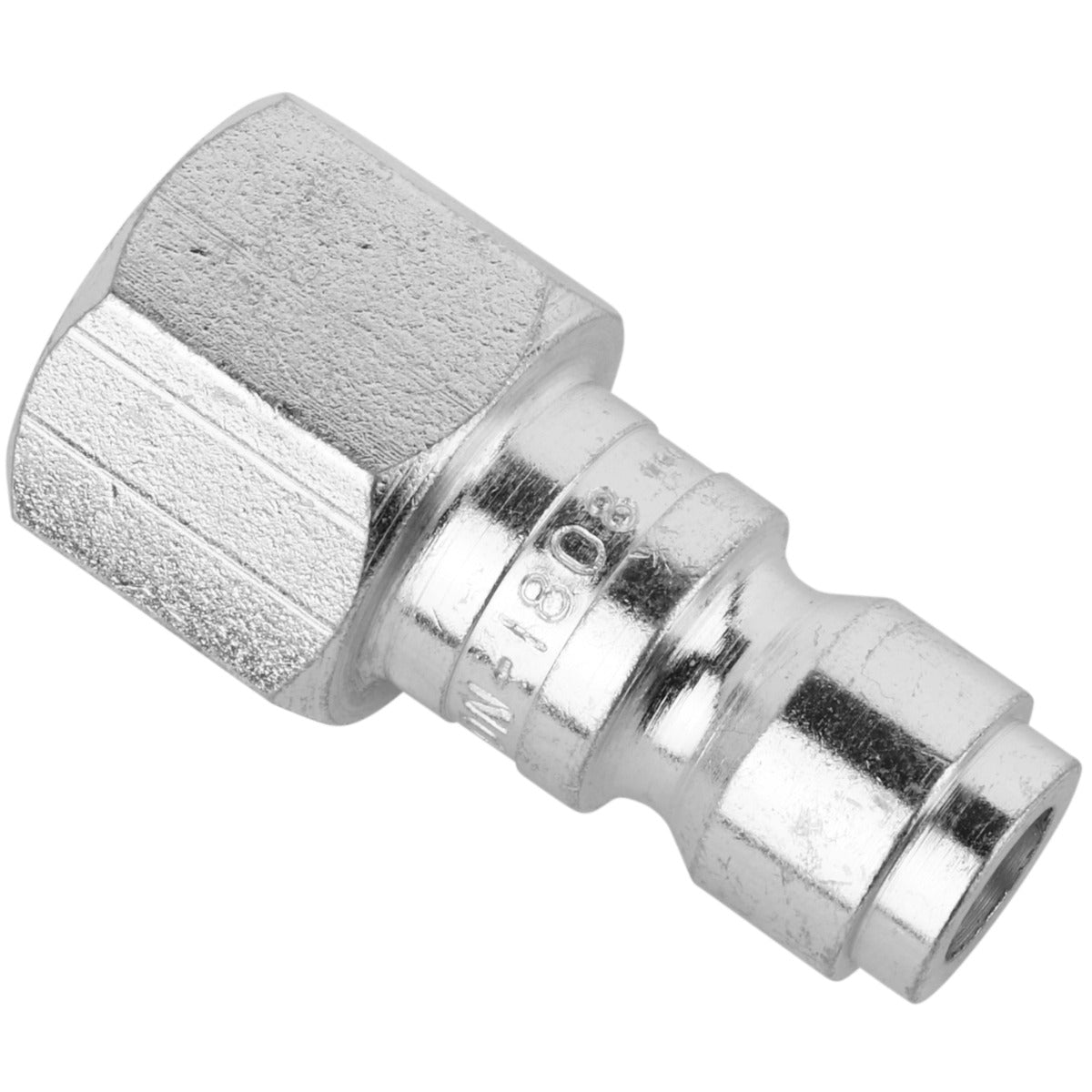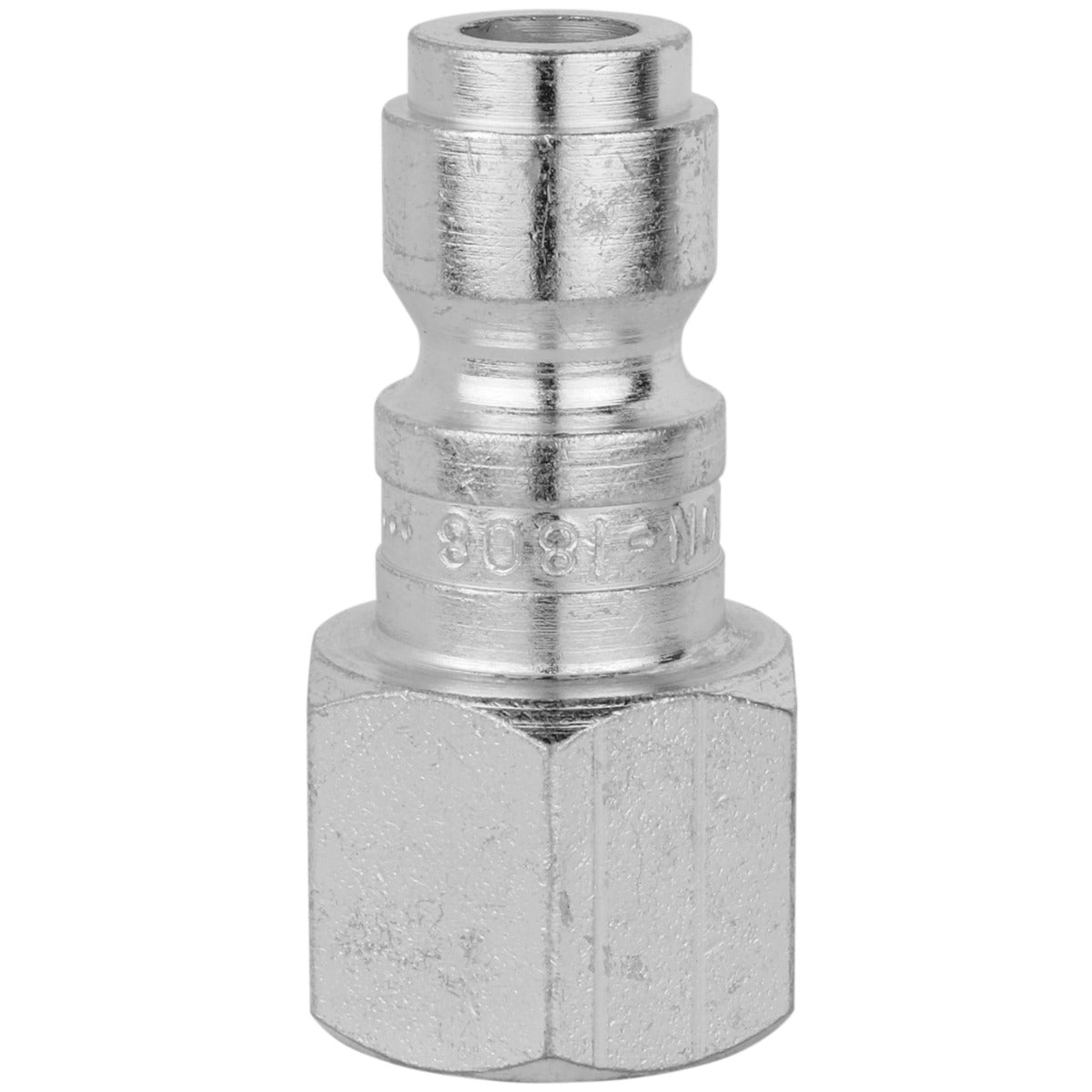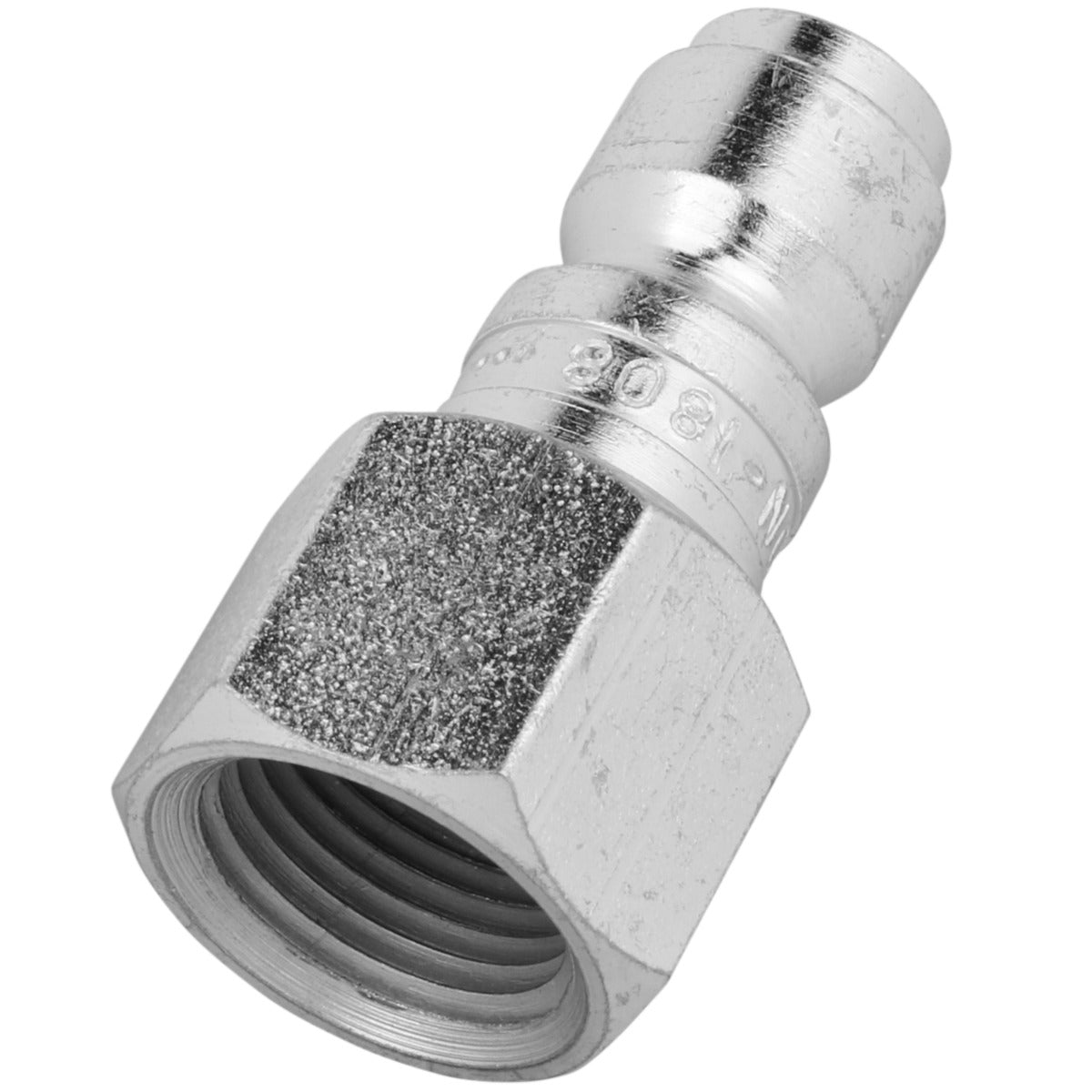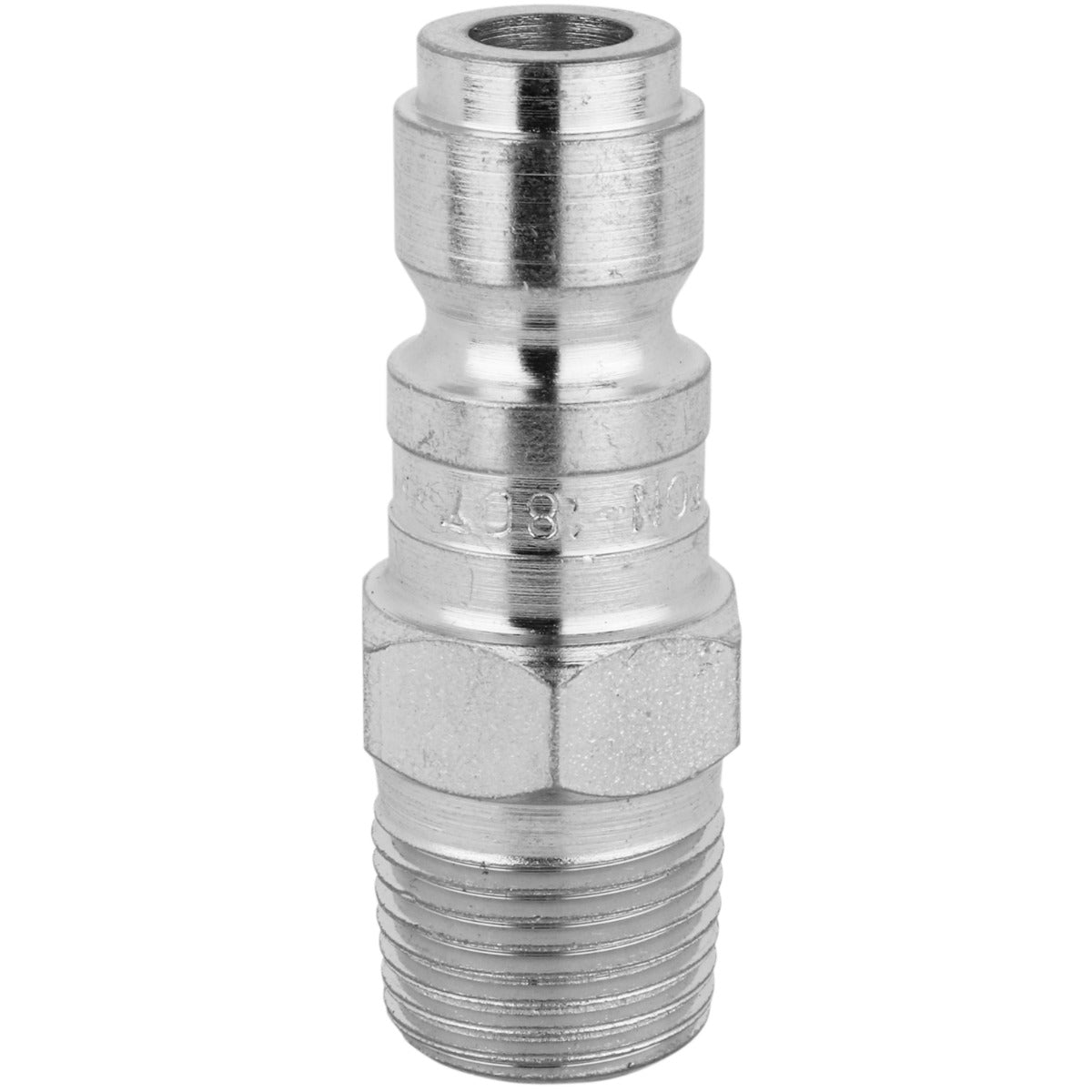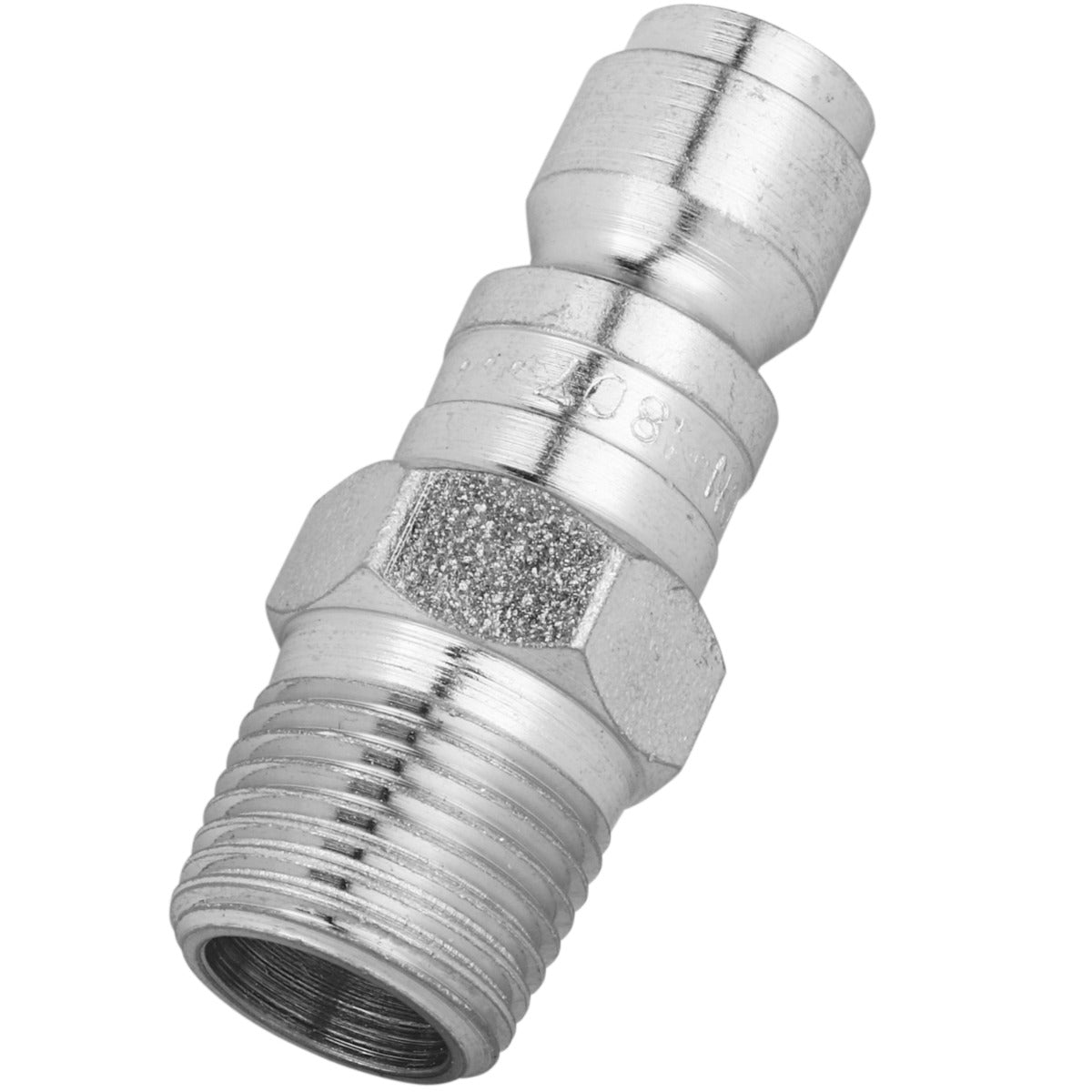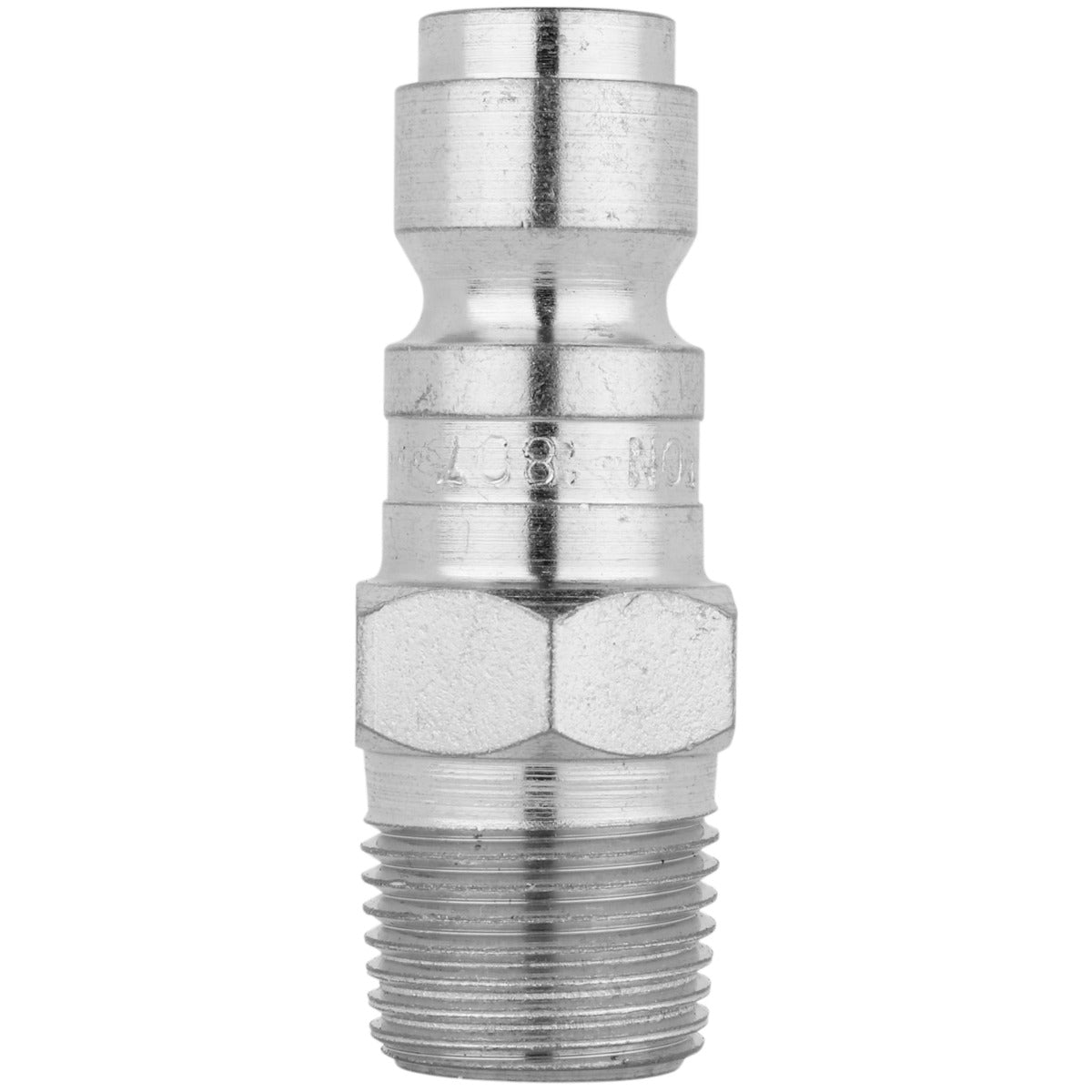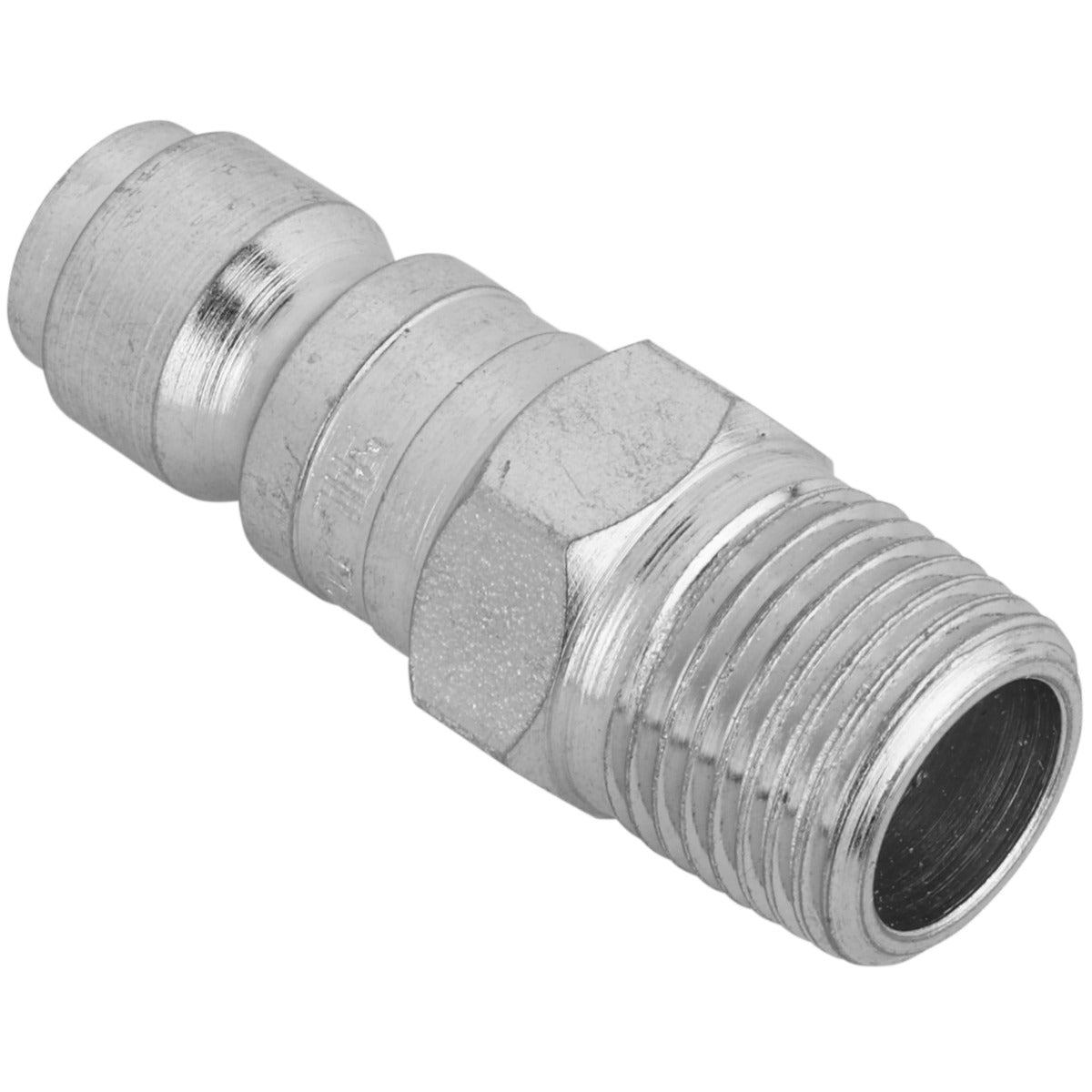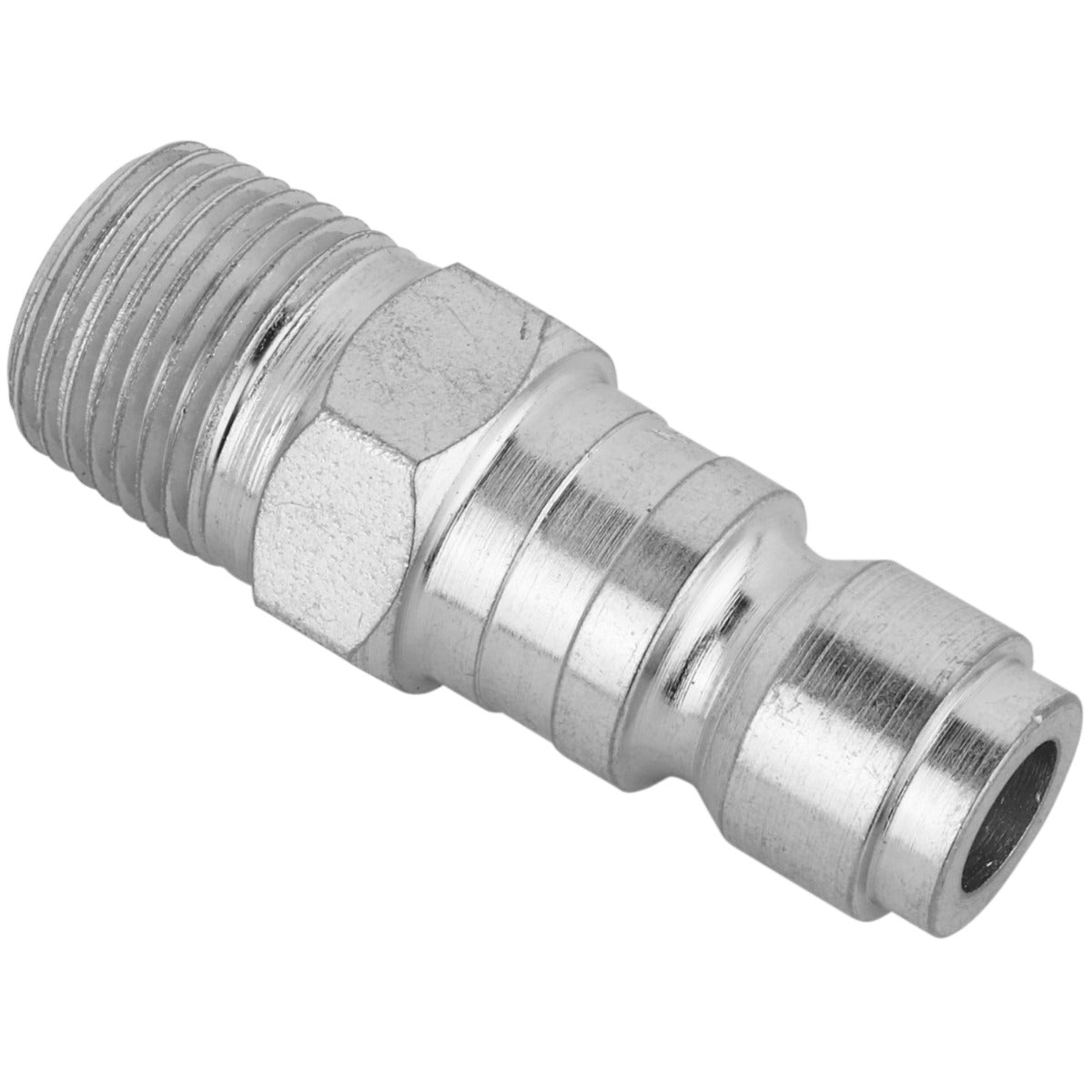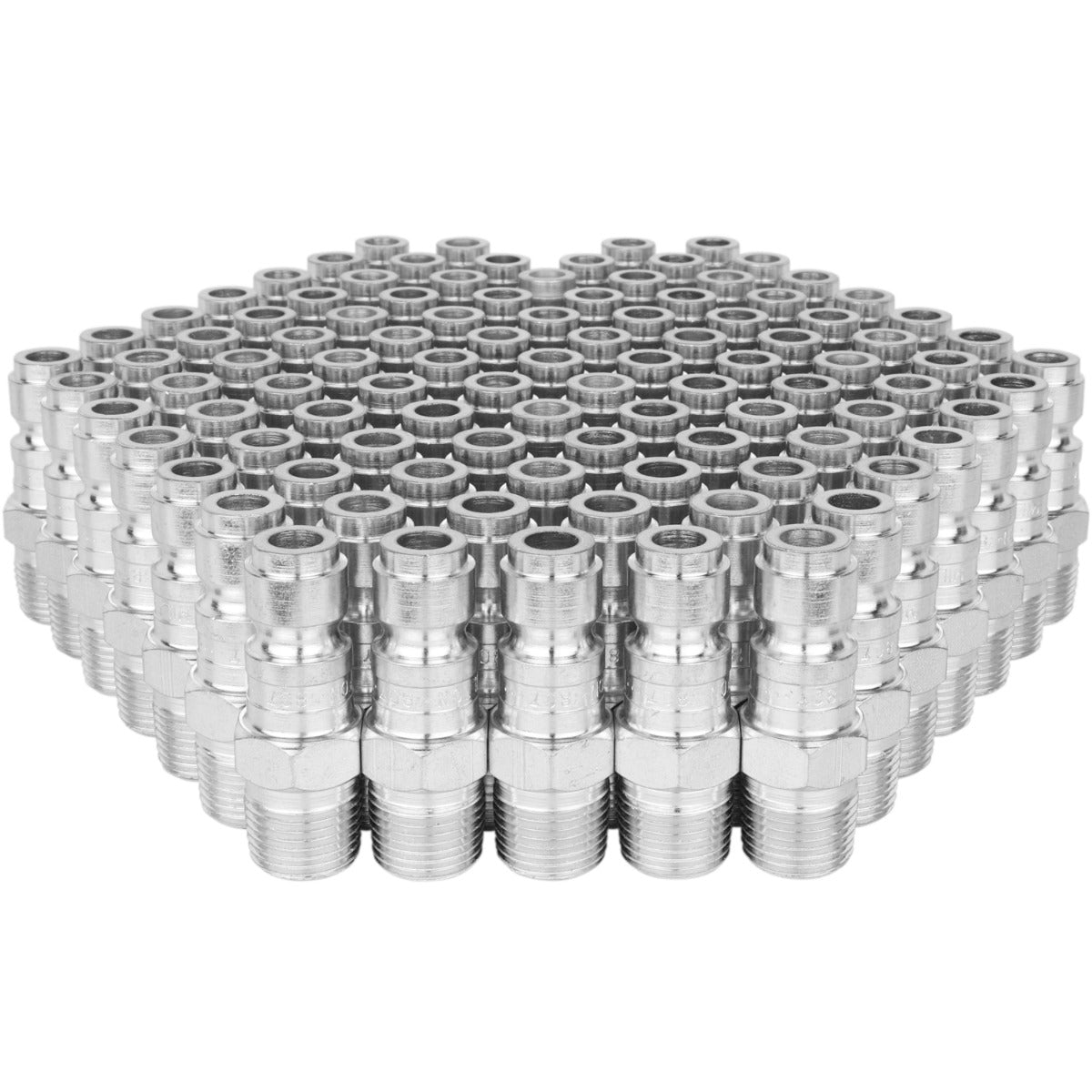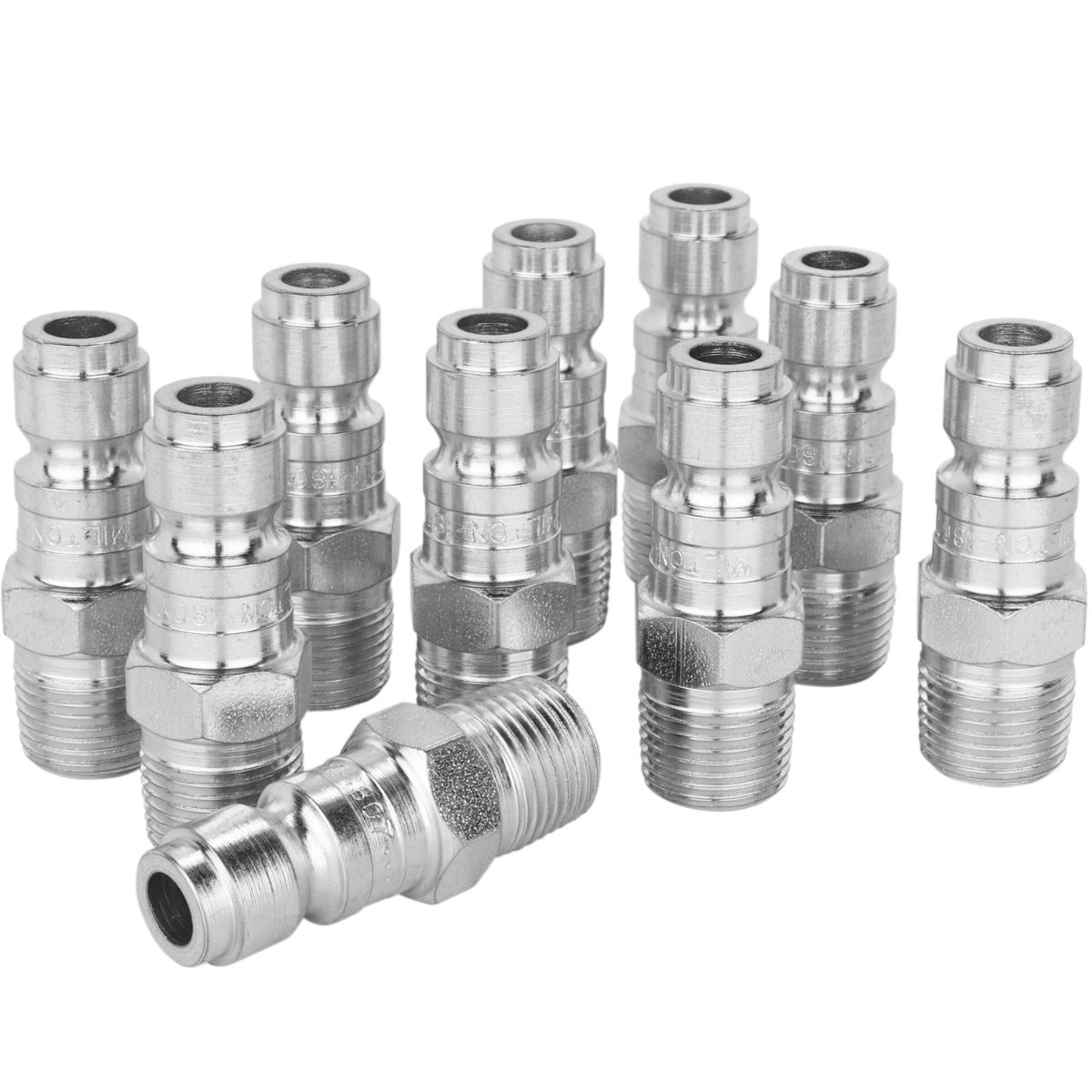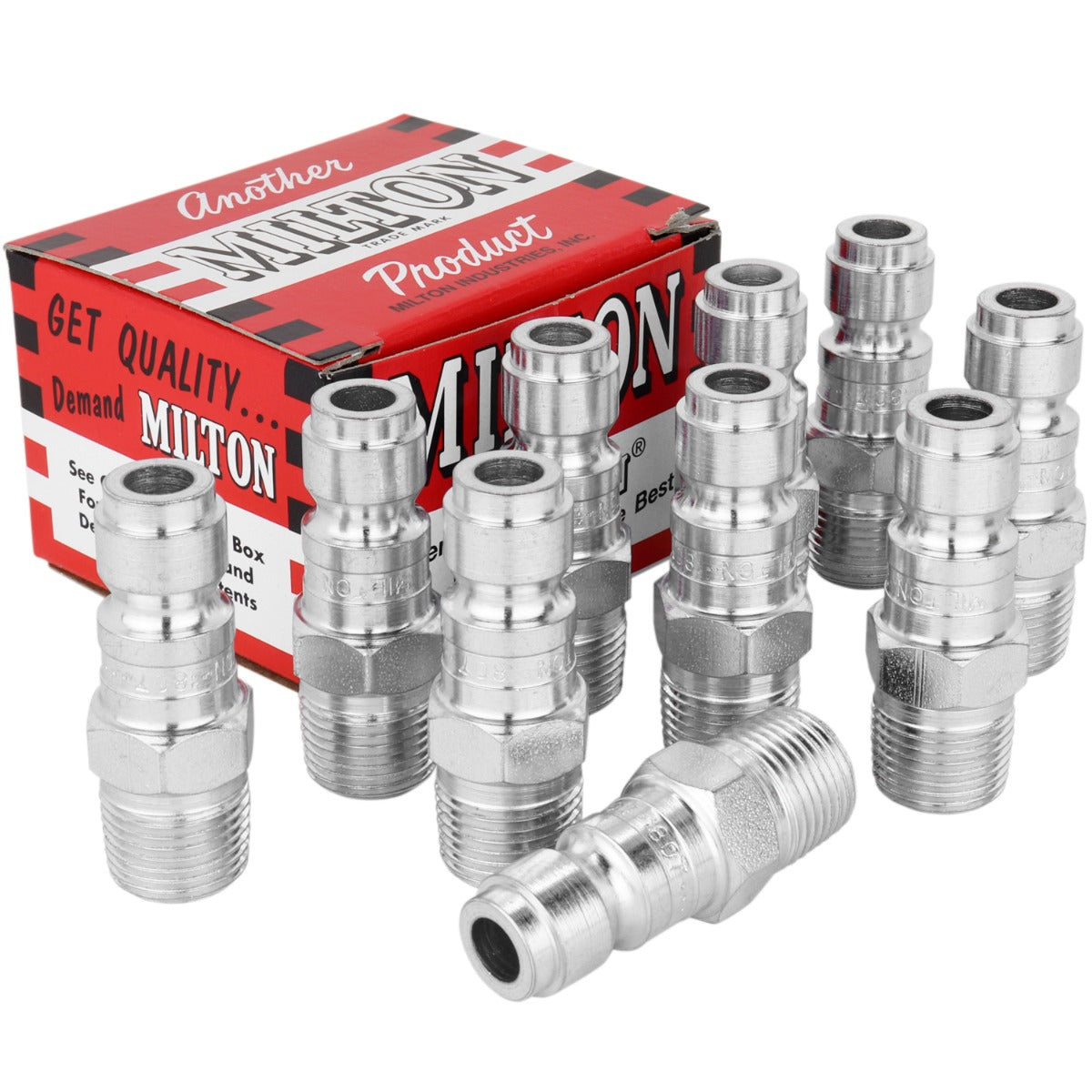Save:### P-Style Plug

Milton Industries Inc.®

Part #: S-1810W
UPC Code: 30937400482
UPC Code: 30937400482
UPC Code: 30937306630
UPC Code: 30937304896
UPC Code: 30937400116
UPC Code: 30937306623
UPC Code: 30937304889
UPC Code: 30937400116
UPC Code: 30937306616
UPC Code: 30937304872
UPC Code: 30937400475
UPC Code: 30937400475
UPC Code: 30937400109
UPC Code: 30937400109
UPC Code: 30937306593
UPC Code: 30937304858
Number of Items: 1
Number of Items: 10
Number of Items: 100
Number of Items: 10
Number of Items: 10
Number of Items: 100
Number of Items: 10
Number of Items: 1
Number of Items: 100
Number of Items: 10
Number of Items: 1
Number of Items: 10
Number of Items: 1
Number of Items: 10
Number of Items: 100
Number of Items: 10
• Maximum of 300 pounds per square inch
• Air flow of 68 standard cubic feet per minute
• Maximum temperature of 250 degrees Fahrenheit
• Maximum of 300 pounds per square inch
• Air flow of 68 standard cubic feet per minute
• Maximum temperature of 250 degrees Fahrenheit
• Maximum of 300 pounds per square inch
• Air flow of 68 standard cubic feet per minute
• Maximum temperature of 250 degrees Fahrenheit
• Maximum of 300 pounds per square inch
• Air flow of 68 standard cubic feet per minute
• Maximum temperature of 250 degrees Fahrenheit
• Maximum of 300 pounds per square inch
• Air flow of 68 standard cubic feet per minute
• Maximum temperature of 250 degrees Fahrenheit
• Maximum of 300 pounds per square inch
• Air flow of 68 standard cubic feet per minute
• Maximum temperature of 250 degrees Fahrenheit
• Maximum of 300 pounds per square inch
• Air flow of 68 standard cubic feet per minute
• Maximum temperature of 250 degrees Fahrenheit
• Maximum of 300 pounds per square inch
• Air flow of 68 standard cubic feet per minute
• Maximum temperature of 250 degrees Fahrenheit
• Maximum of 300 pounds per square inch
• Air flow of 68 standard cubic feet per minute
• Maximum temperature of 250 degrees Fahrenheit
• Maximum of 300 pounds per square inch
• Air flow of 68 standard cubic feet per minute
• Maximum temperature of 250 degrees Fahrenheit
• Maximum of 300 pounds per square inch
• Air flow of 68 standard cubic feet per minute
• Maximum temperature of 250 degrees Fahrenheit
• Maximum of 300 pounds per square inch
• Air flow of 68 standard cubic feet per minute
• Maximum temperature of 250 degrees Fahrenheit
• Maximum of 300 pounds per square inch
• Air flow of 68 standard cubic feet per minute
• Maximum temperature of 250 degrees Fahrenheit
• Maximum of 300 pounds per square inch
• Air flow of 68 standard cubic feet per minute
• Maximum temperature of 250 degrees Fahrenheit
• Maximum of 300 pounds per square inch
• Air flow of 68 standard cubic feet per minute
• Maximum temperature of 250 degrees Fahrenheit
• Maximum of 300 pounds per square inch
• Air flow of 68 standard cubic feet per minute
• Maximum temperature of 250 degrees Fahrenheit
\$7.52
Save:

### Quantity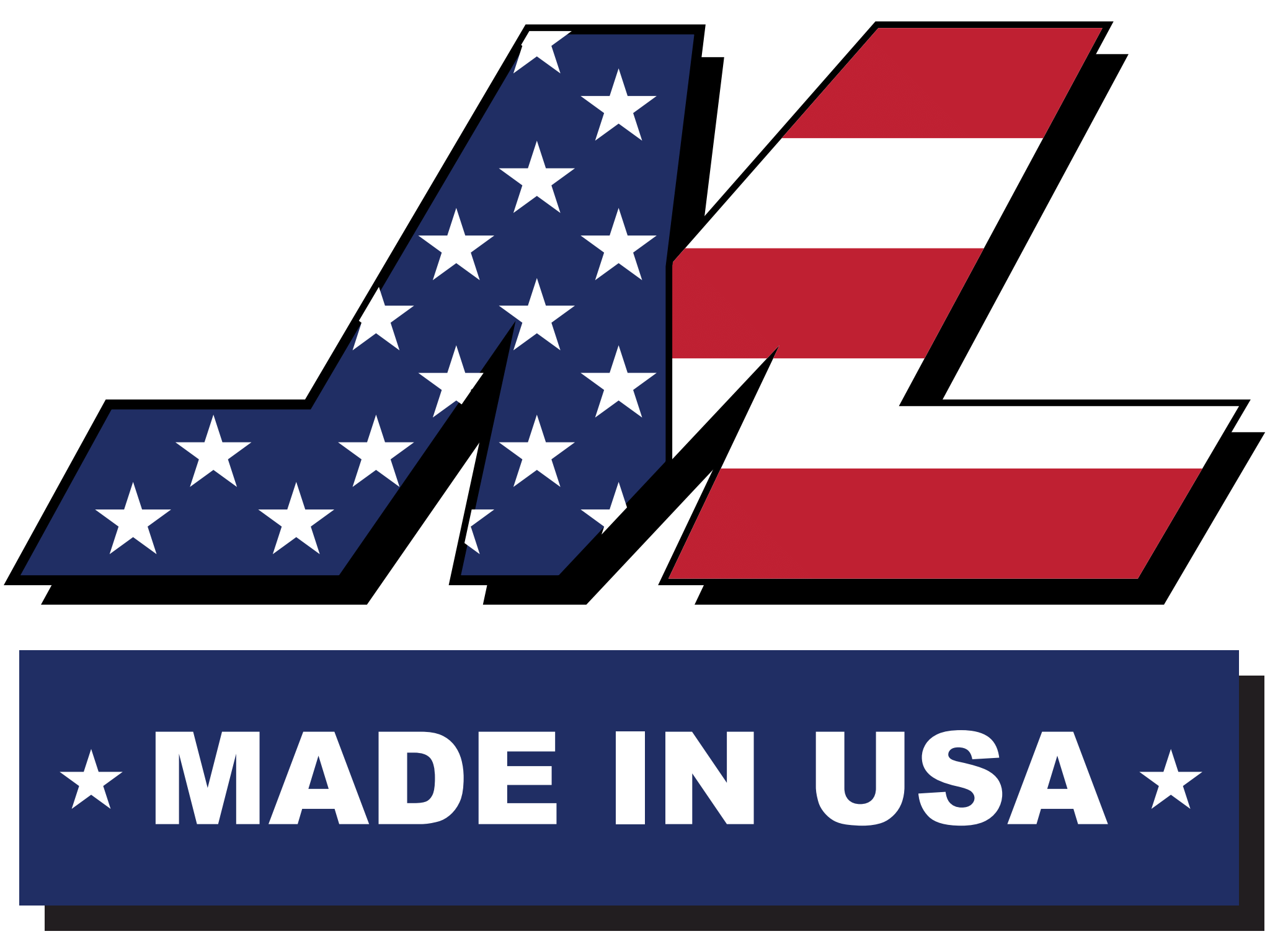### P-Style Plug

MNPT P style plugs are made of case hardened steel and are plated to resist rust. P style plugs offer a 3/8" basic flow size and a maximum pressure of 300 PSI. Air flow of 68 SCFM.

MNPT P style plugs are made of case hardened steel and are plated to resist rust. P style plugs offer a 3/8" basic flow size and a maximum pressure of 300 PSI. Air flow of 68 SCFM.

 Type of Hose : Type of fiiting : Thread Size : SCFM : Air Flow 68 SCFM PSI range :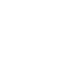### FREE SHIPPING

When you spend \$75+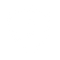### WARRANTY INFO

Offering a range of policies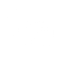### NEED HELP

Contact our support team today### FIND A DISTRIBUTOR

Locate the closest inventory